# ML Aggarwal Solutions for Class 10 Maths Chapter 12 Equation of Straight Line

ML Aggarwal Solutions for Class 10 Maths Chapter 12 Equation of Straight Line aid students for effective preparation for their examinations. The solutions are created by subject matter experts after acquiring a vast knowledge of the concepts present in this chapter. For a better way to learn the concepts, ML Aggarwal Solutions for Class 10 Maths Chapter 12 Equation of Straight Line PDF link is attached here for download.

Chapter 12 of ML Aggarwal Solutions contains problems in determining the equation of a straight line in different forms as in the prescribed textbook. Students can prepare for their examinations in a more effective manner using these ML Aggarwal Solutions which is available for free. The PDF materials are listed on the BYJU’S website in order to promote both online as well as offline mode of learning without any time constraints.

## PDF Solution for ML Aggarwal Class 10 Maths Chapter 12 :-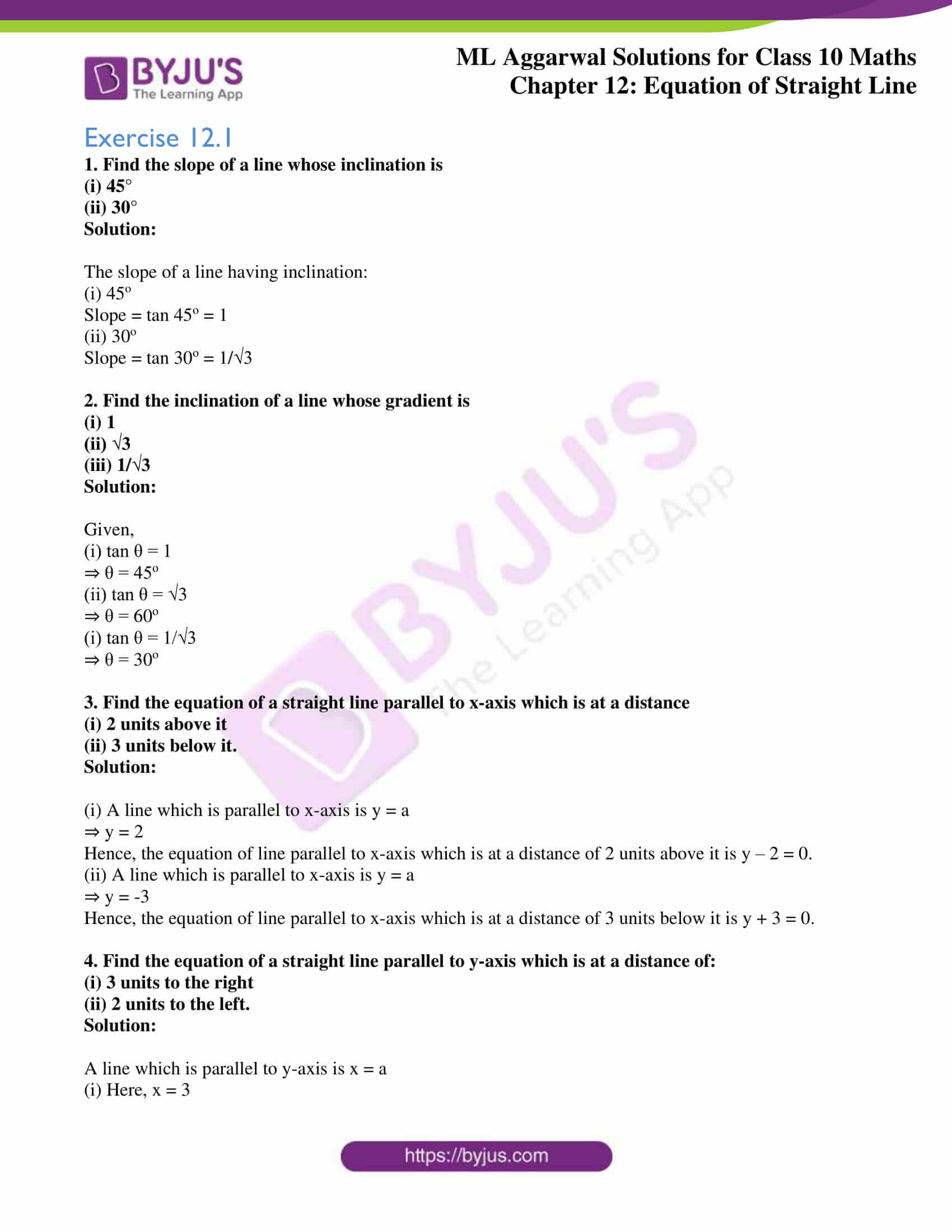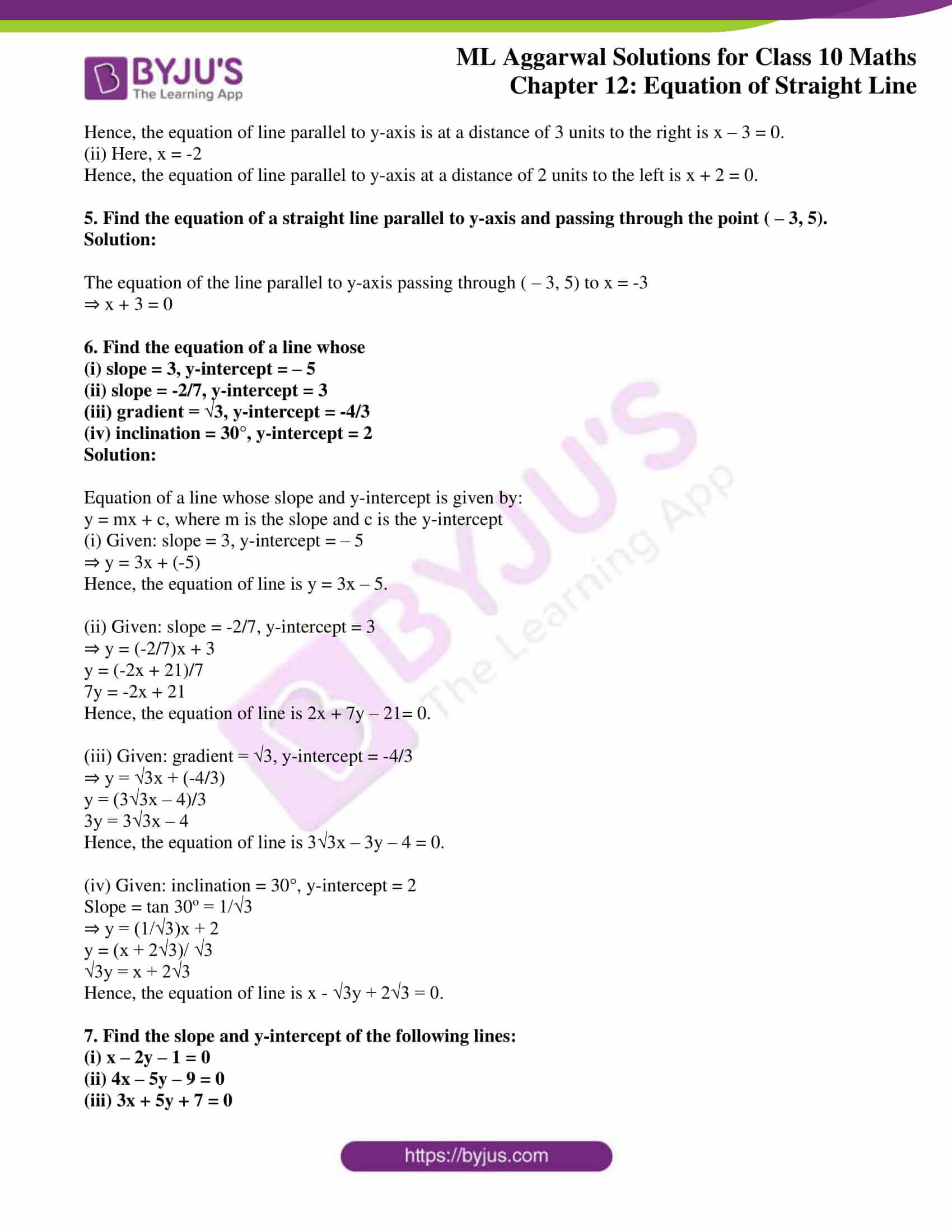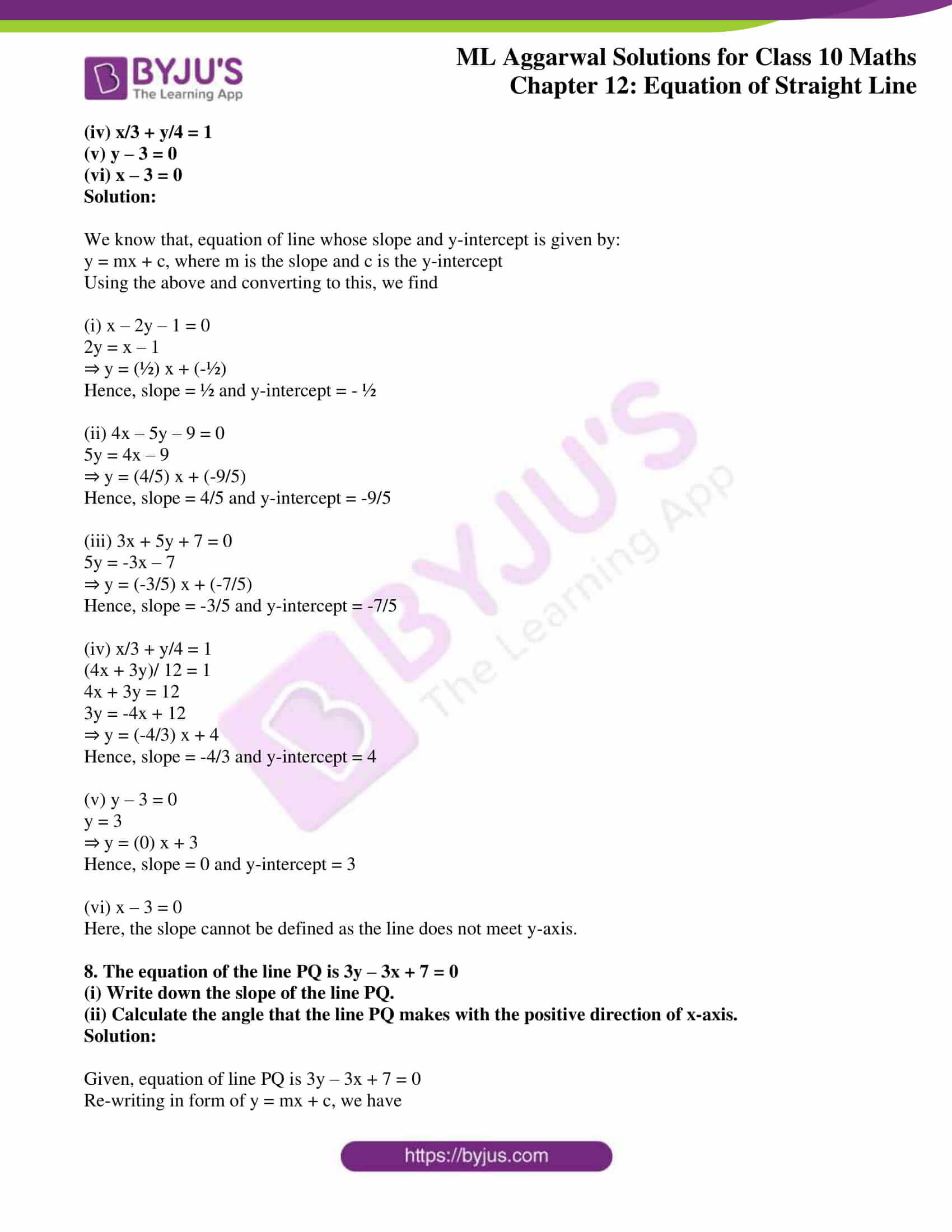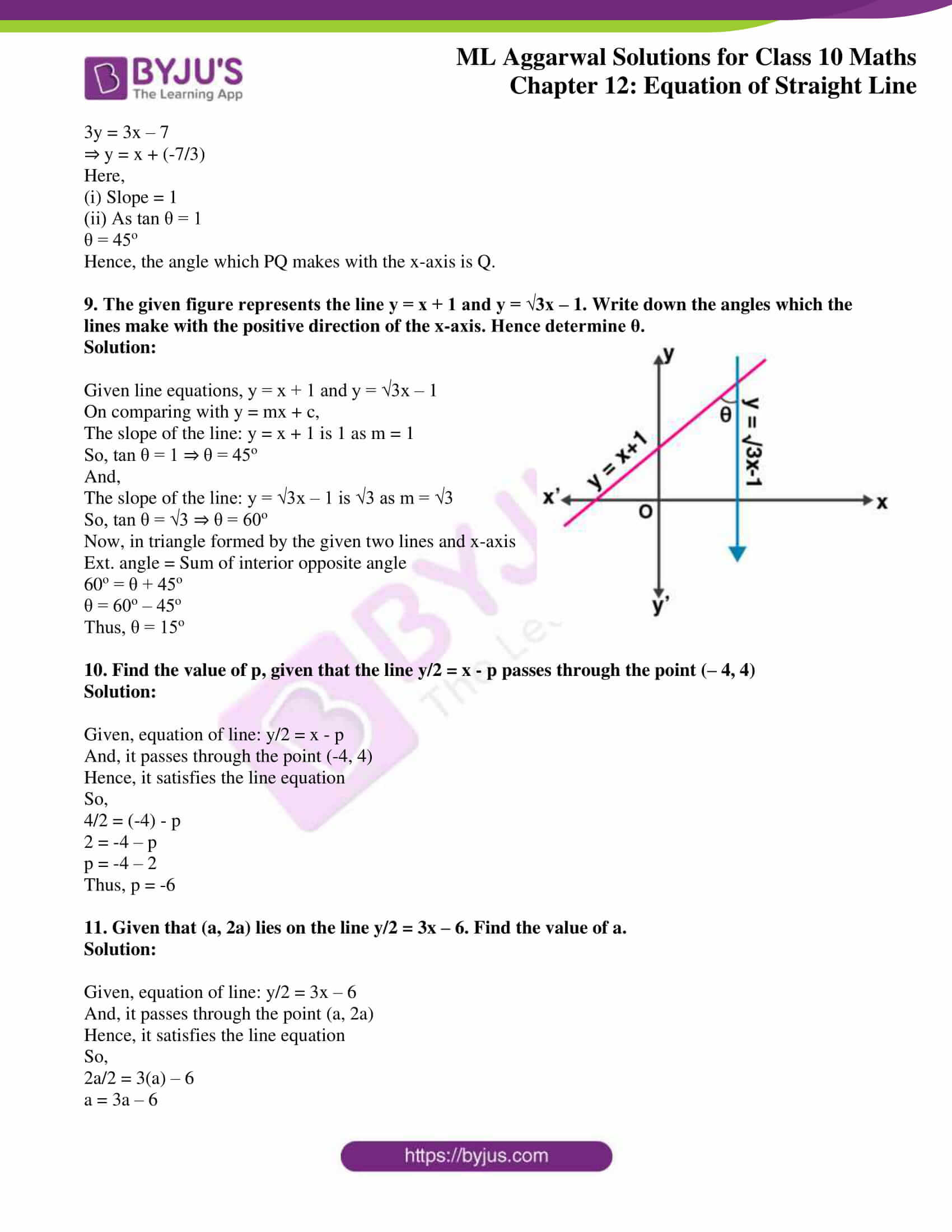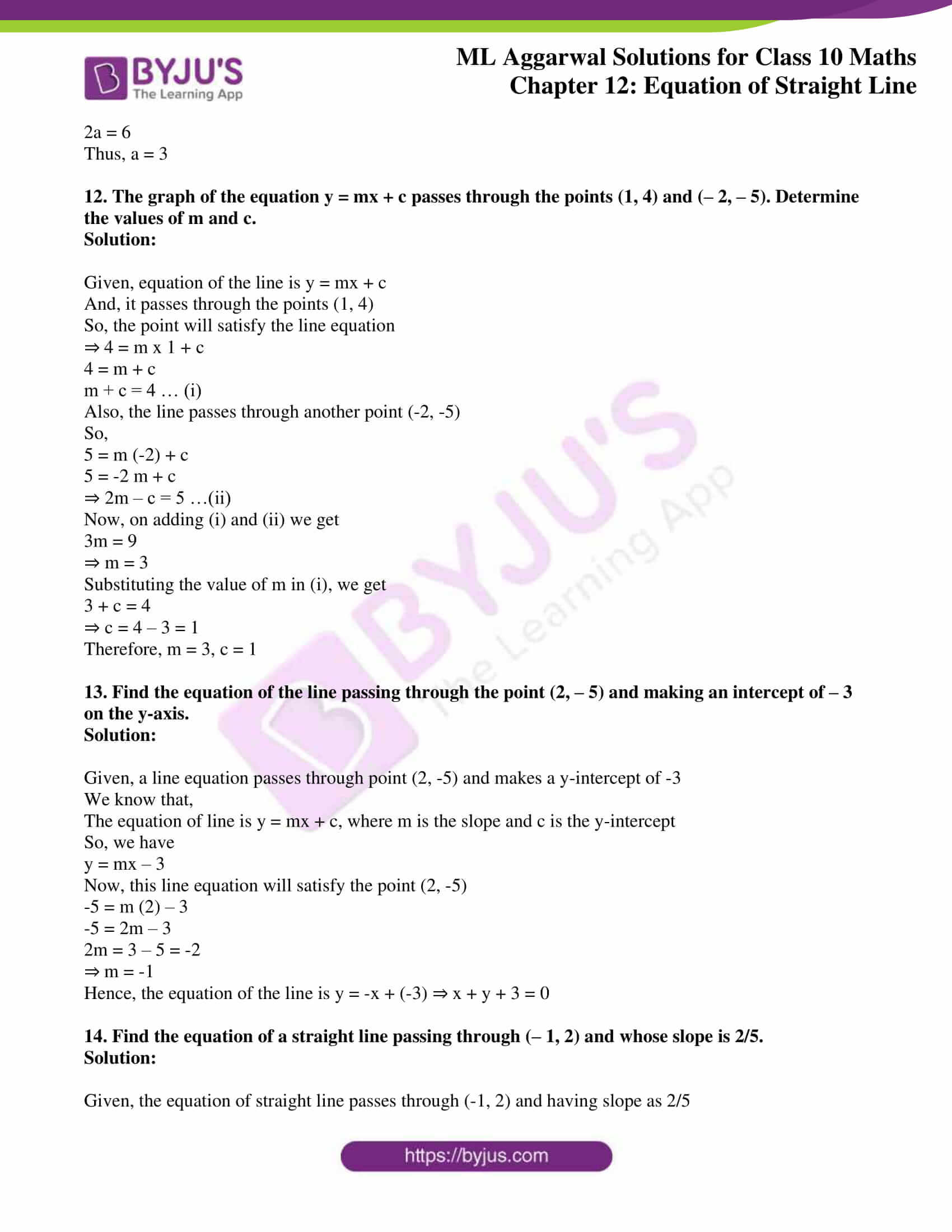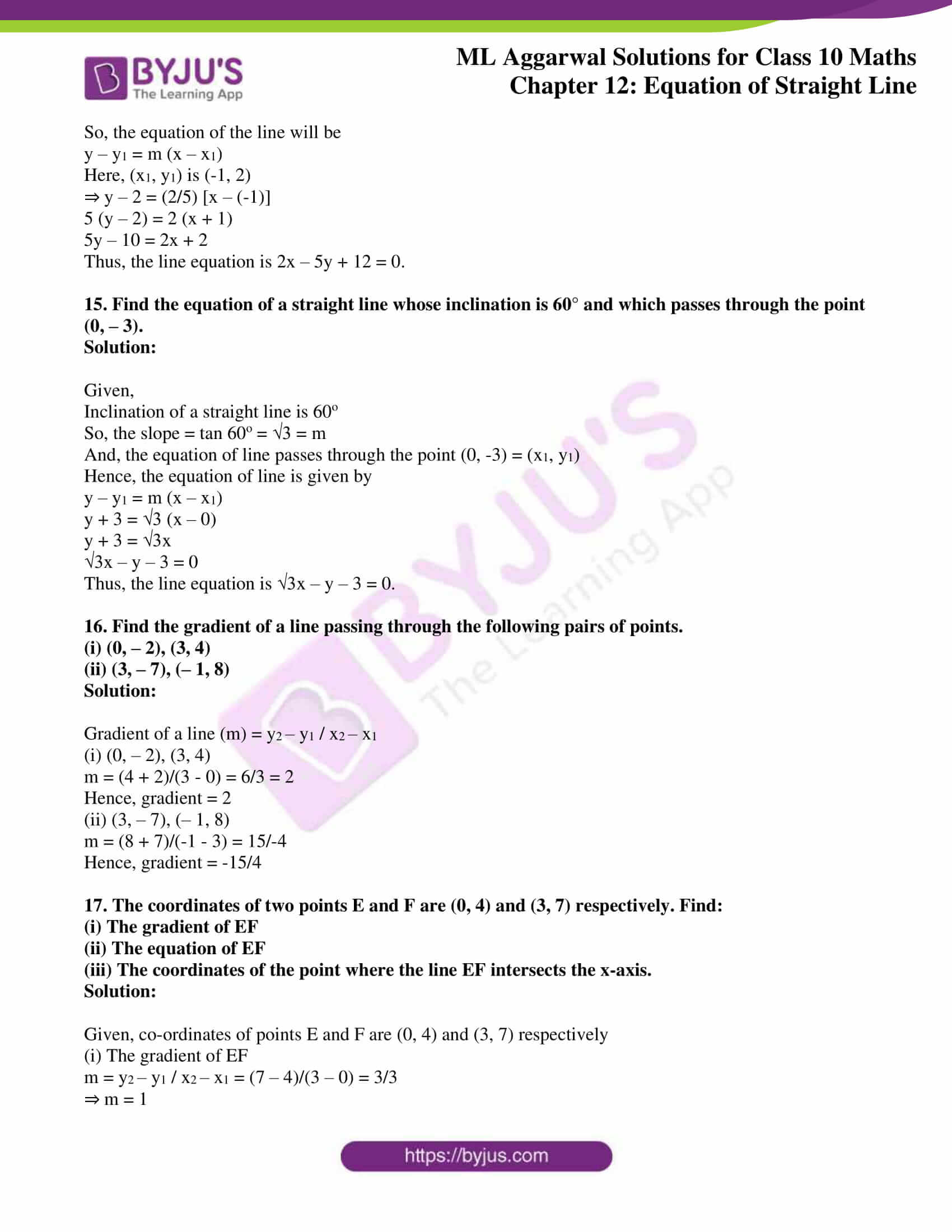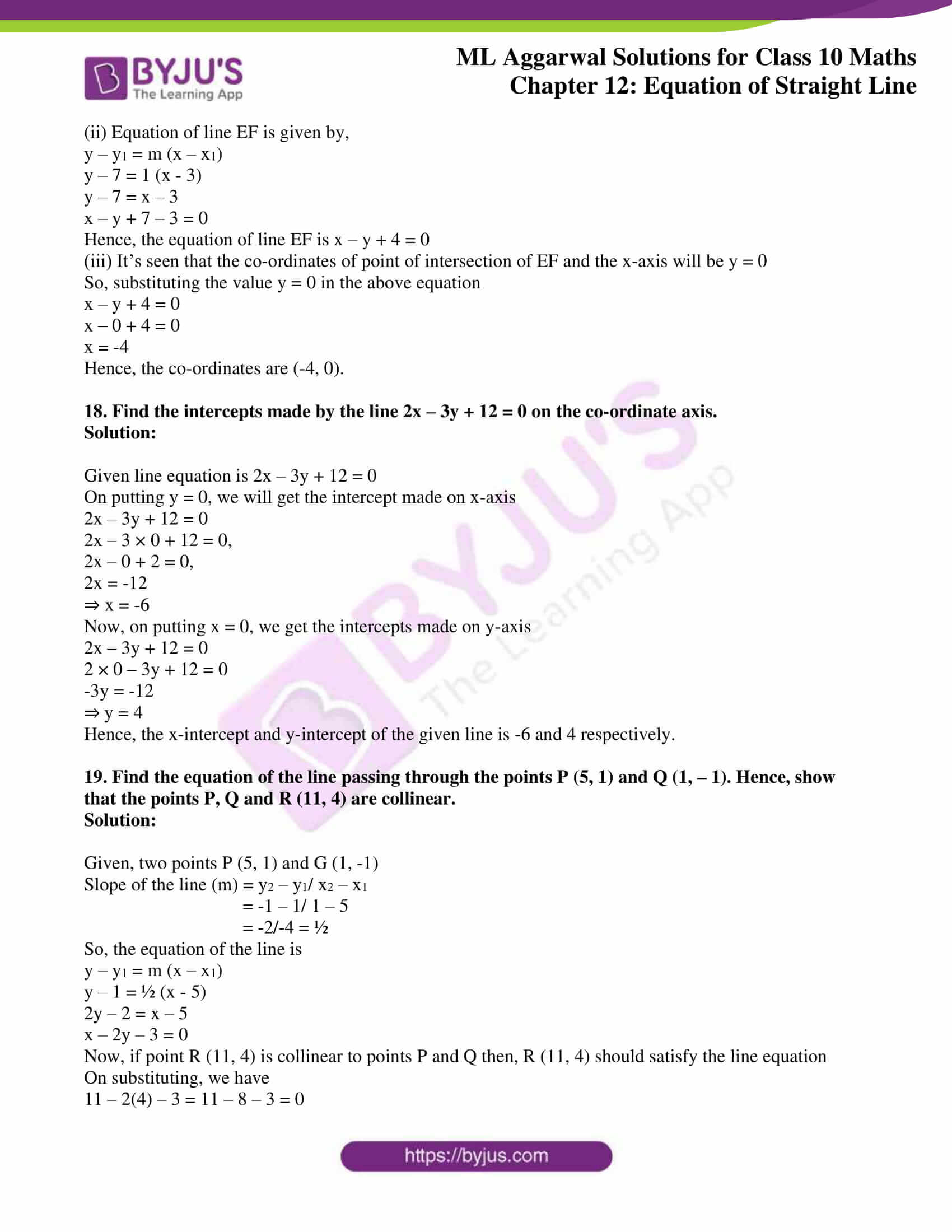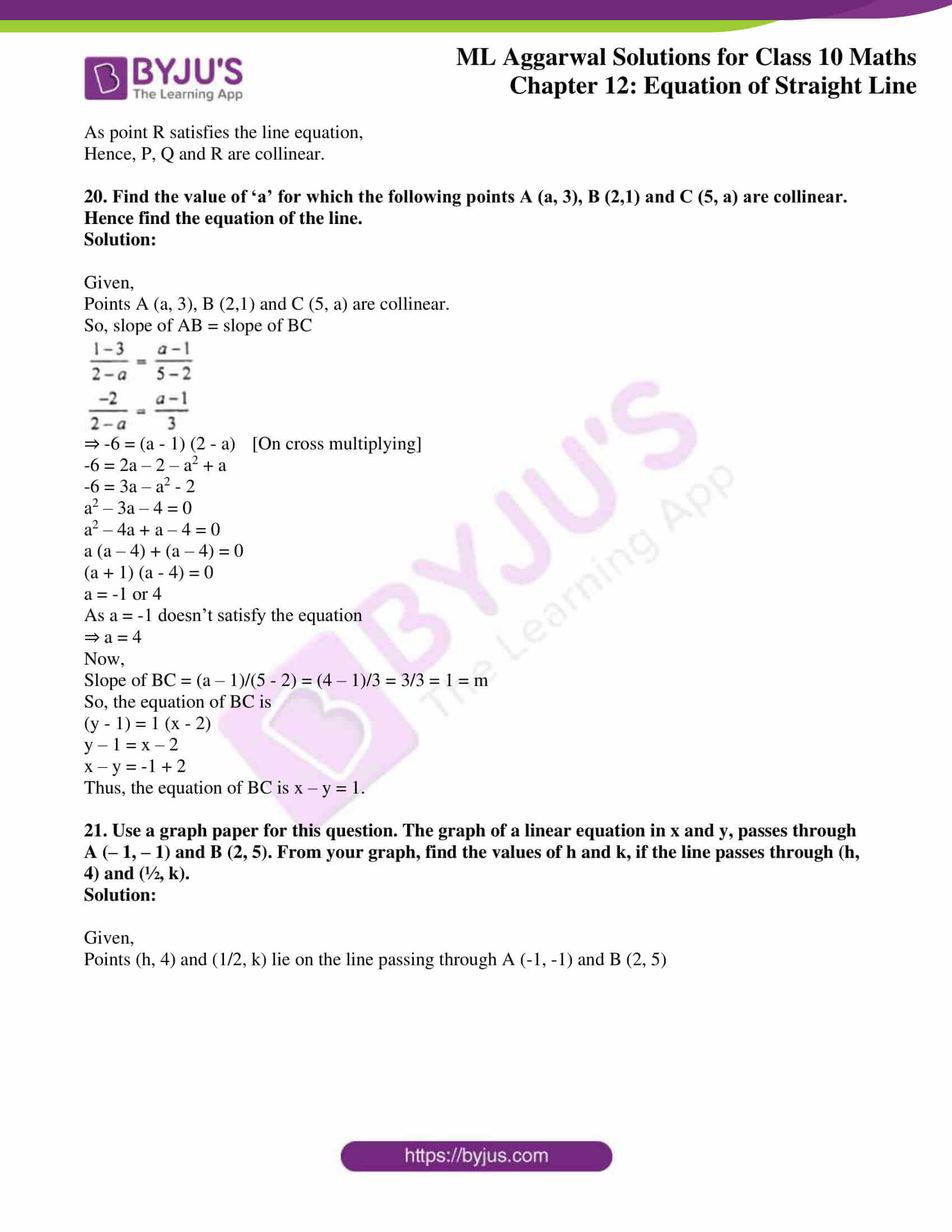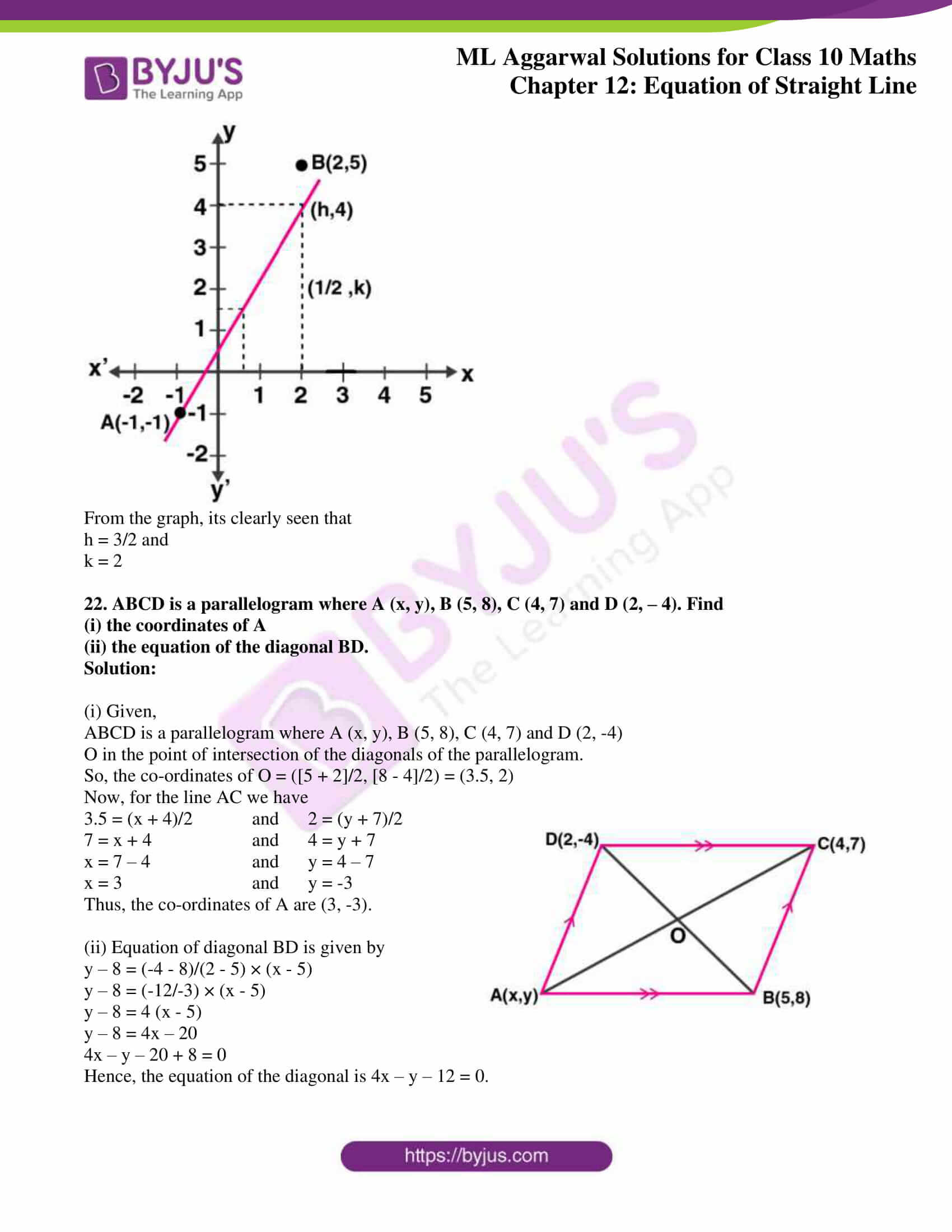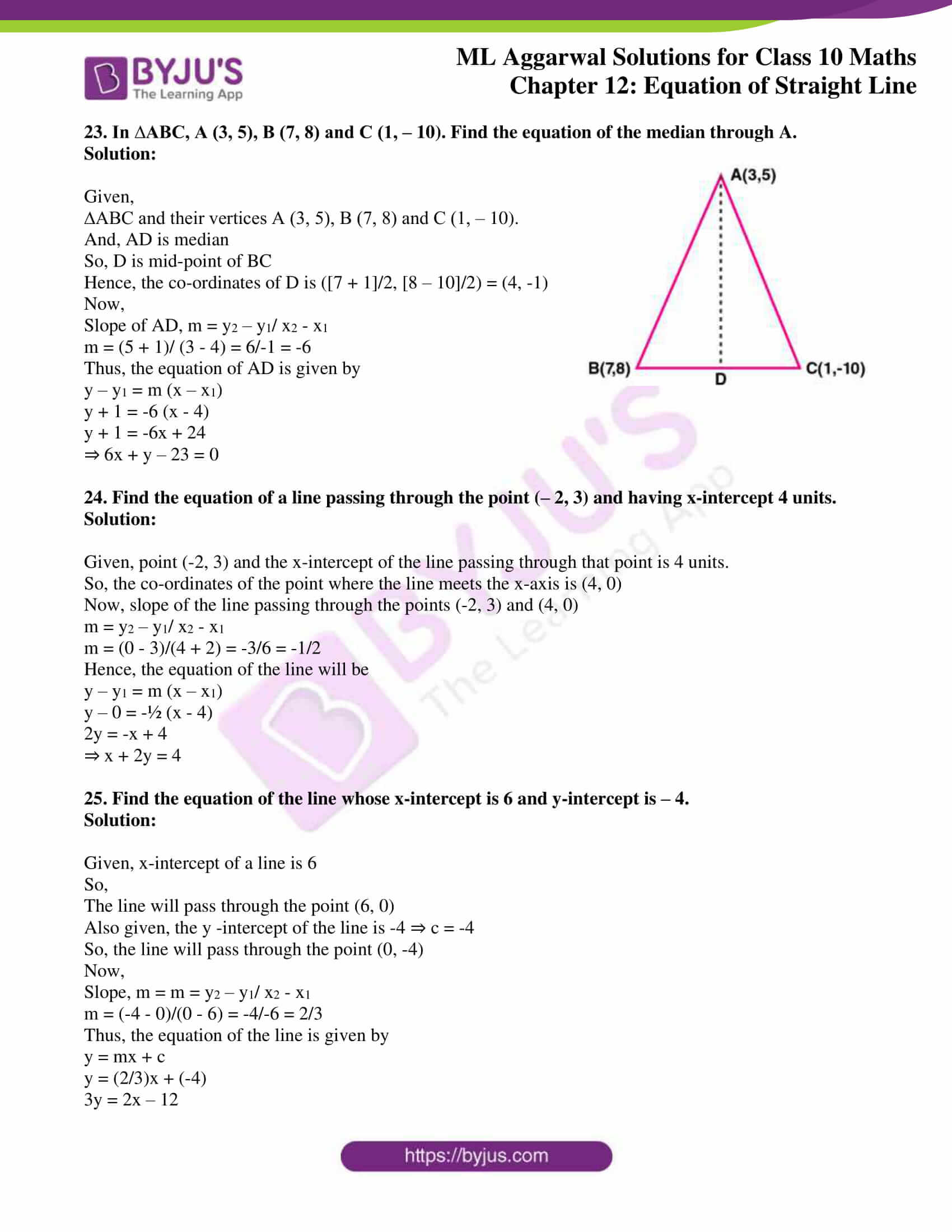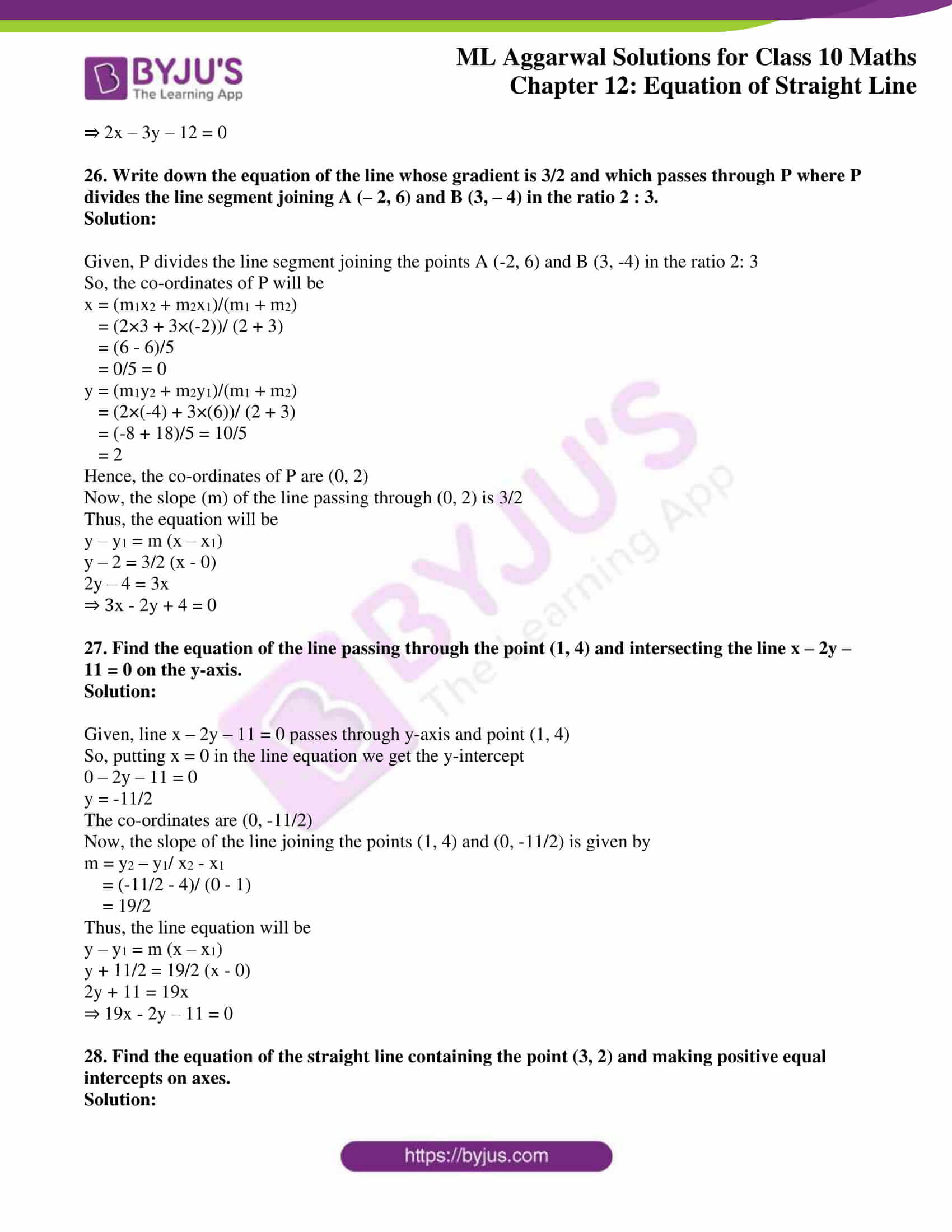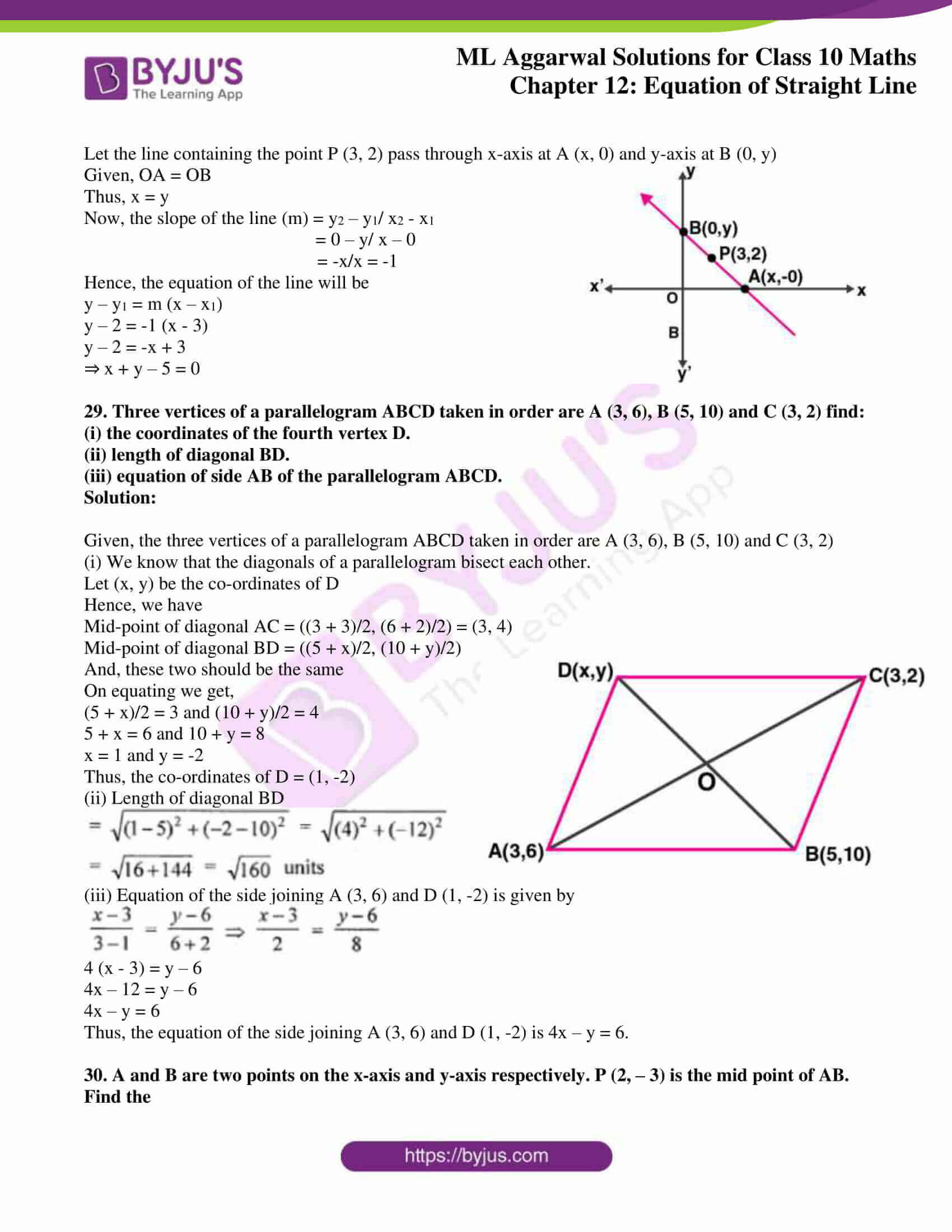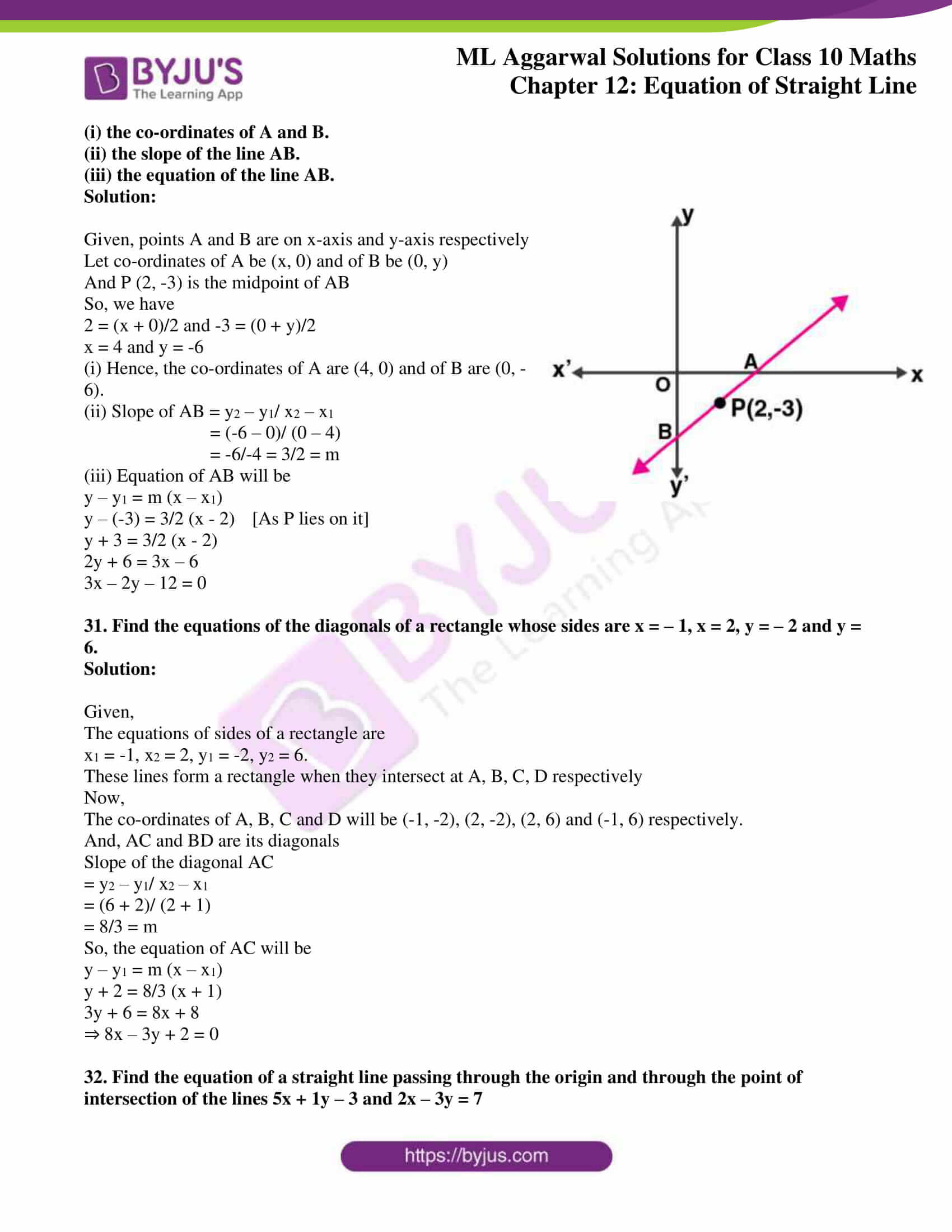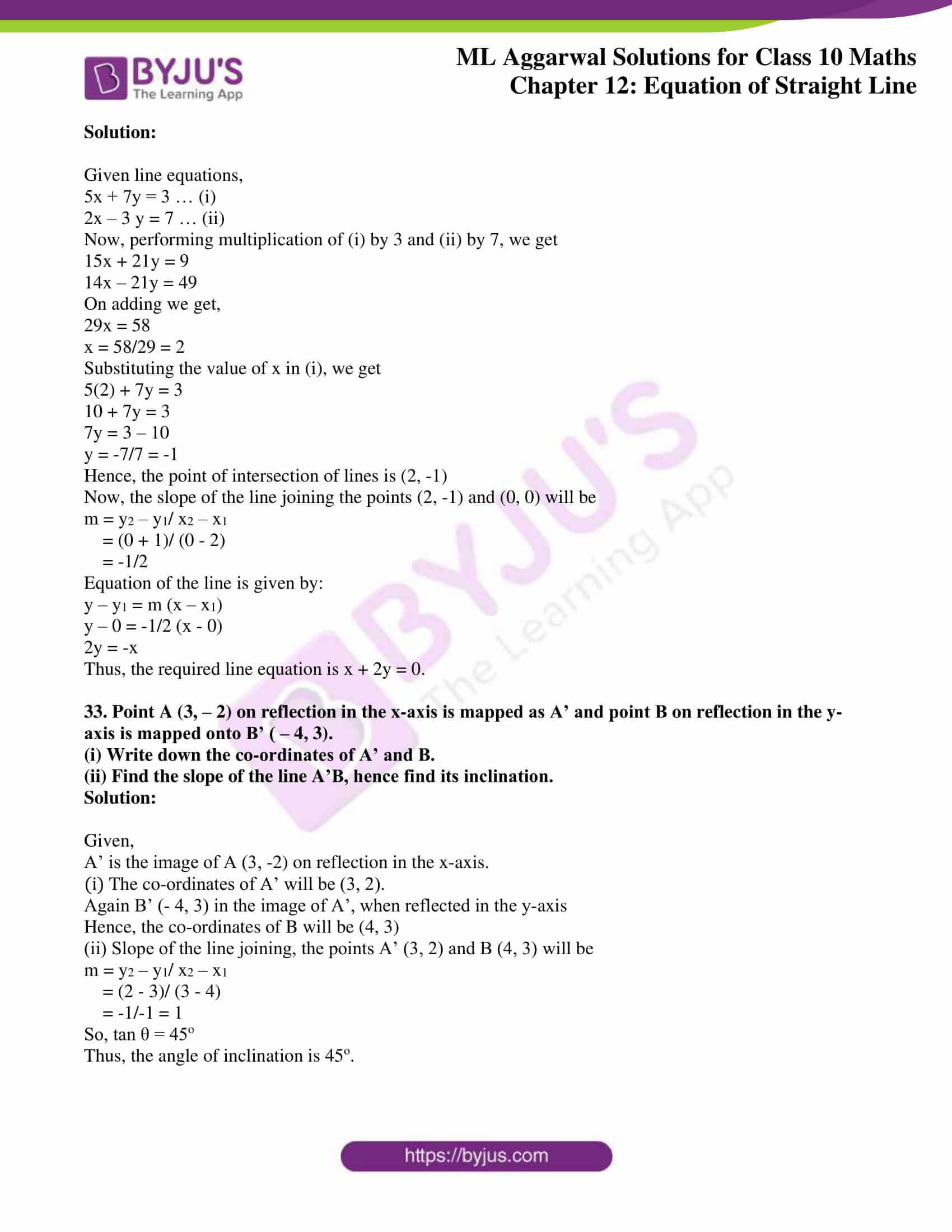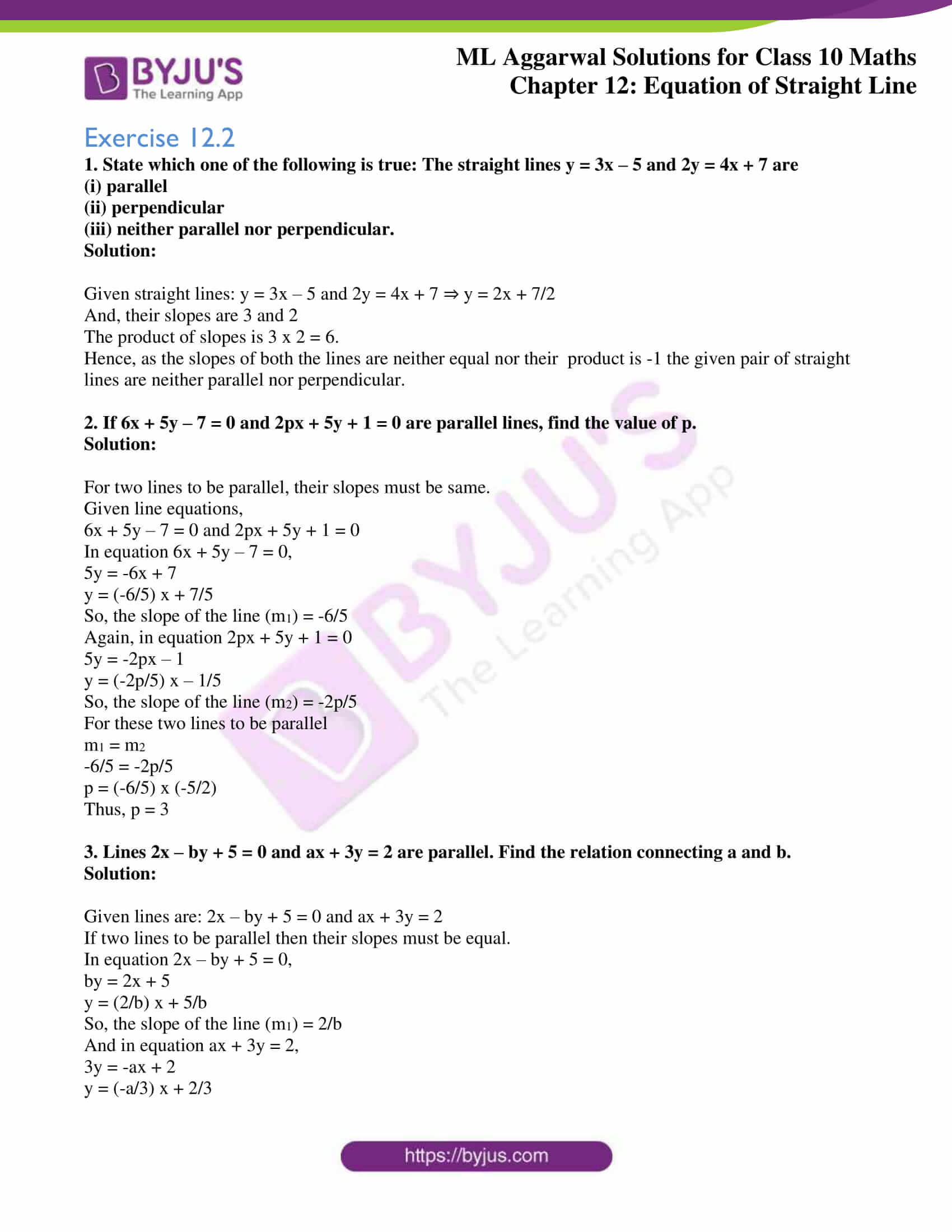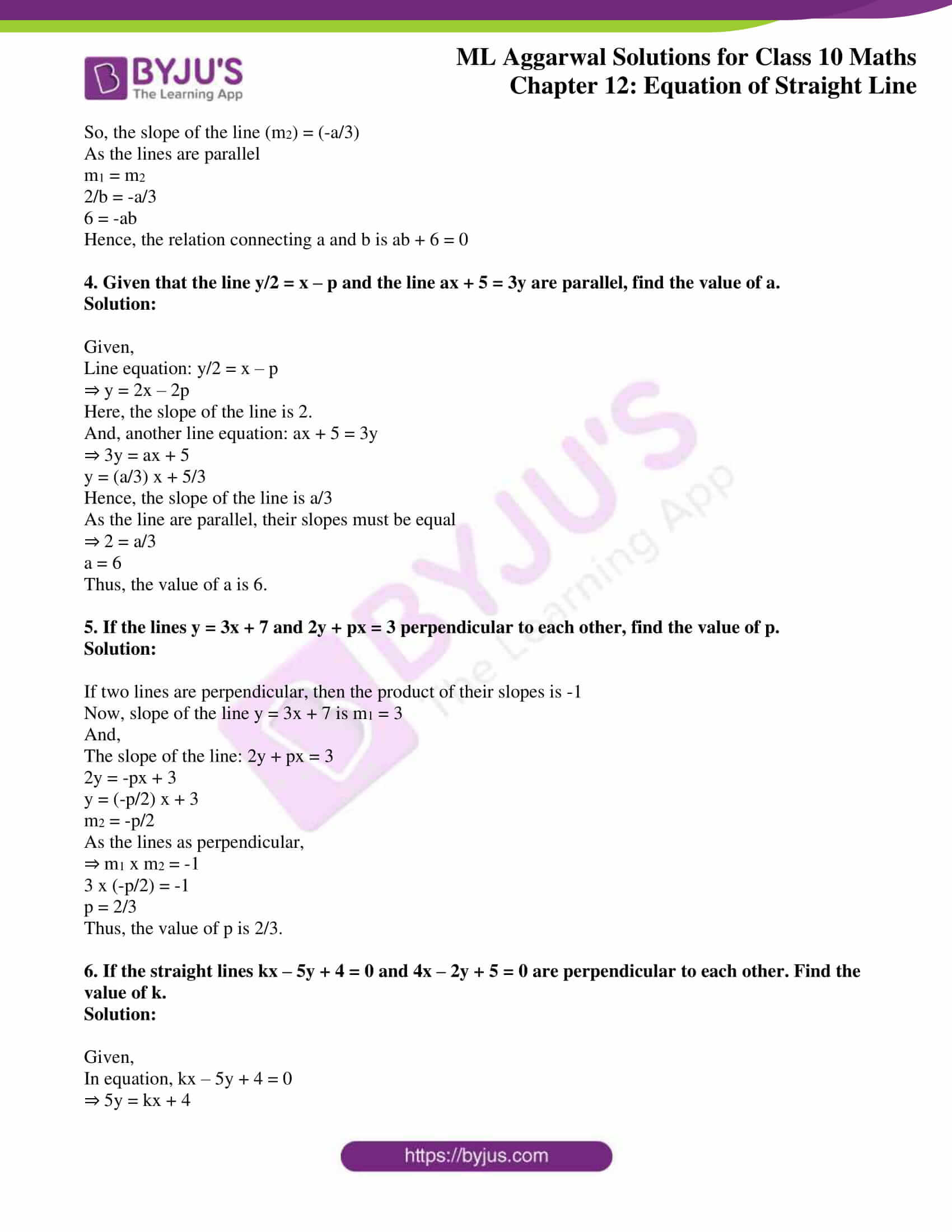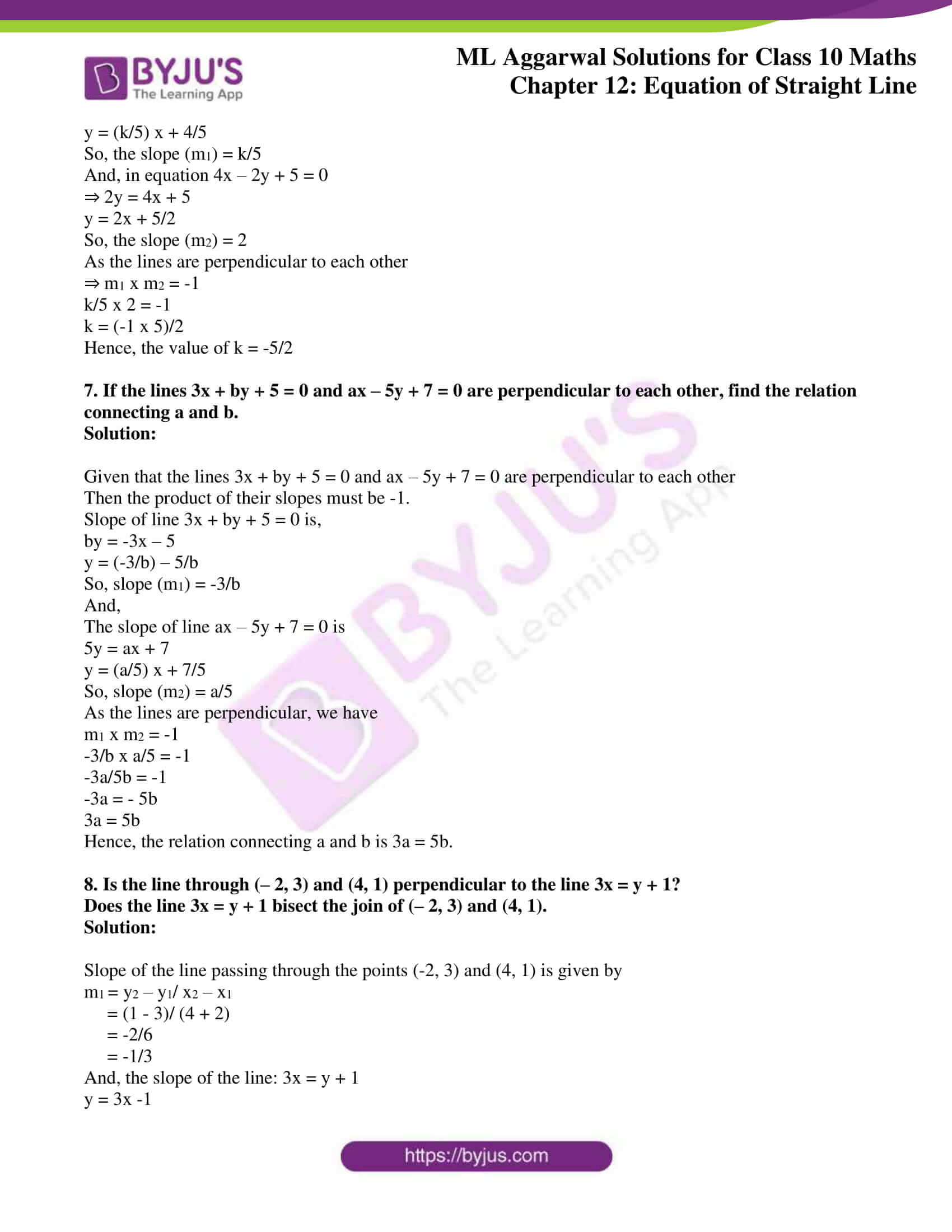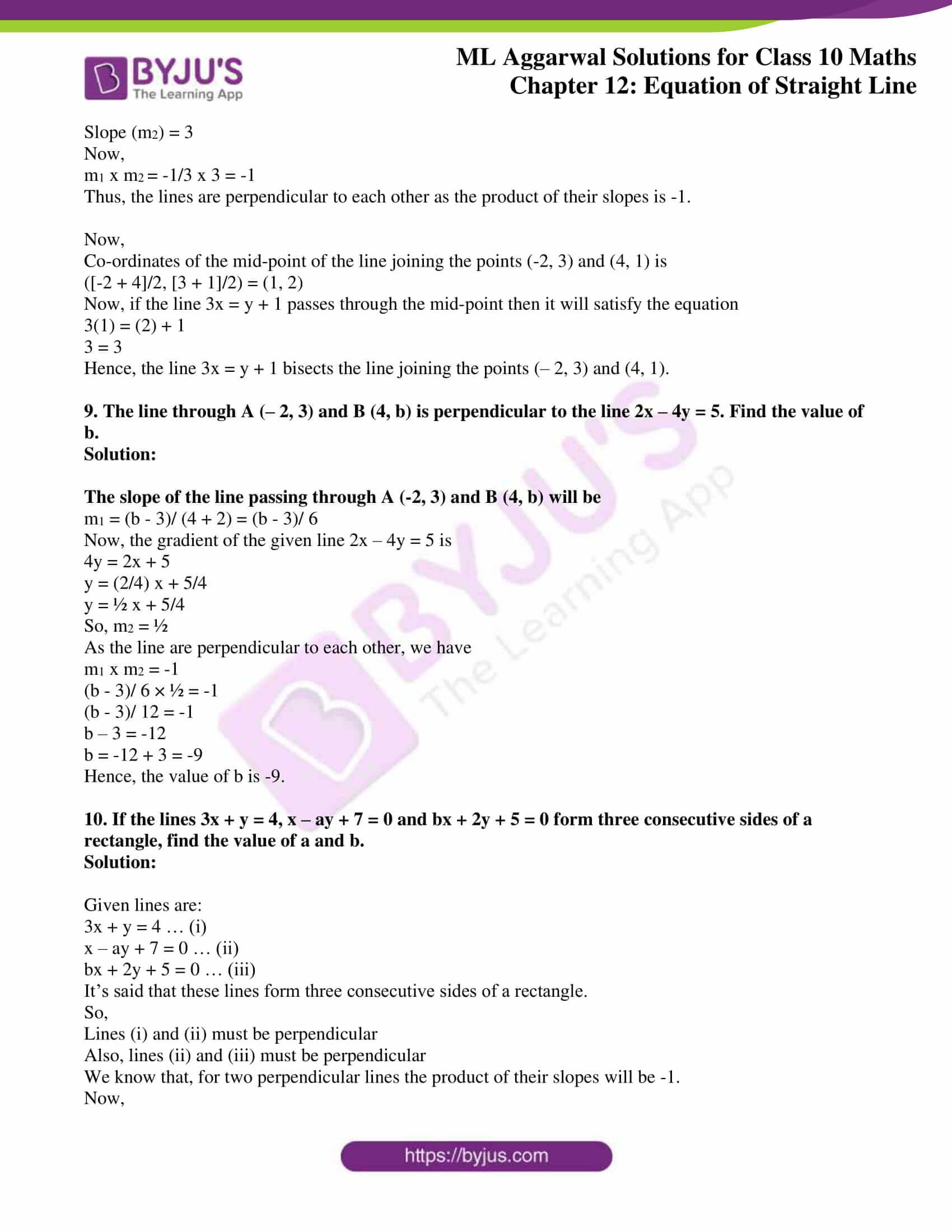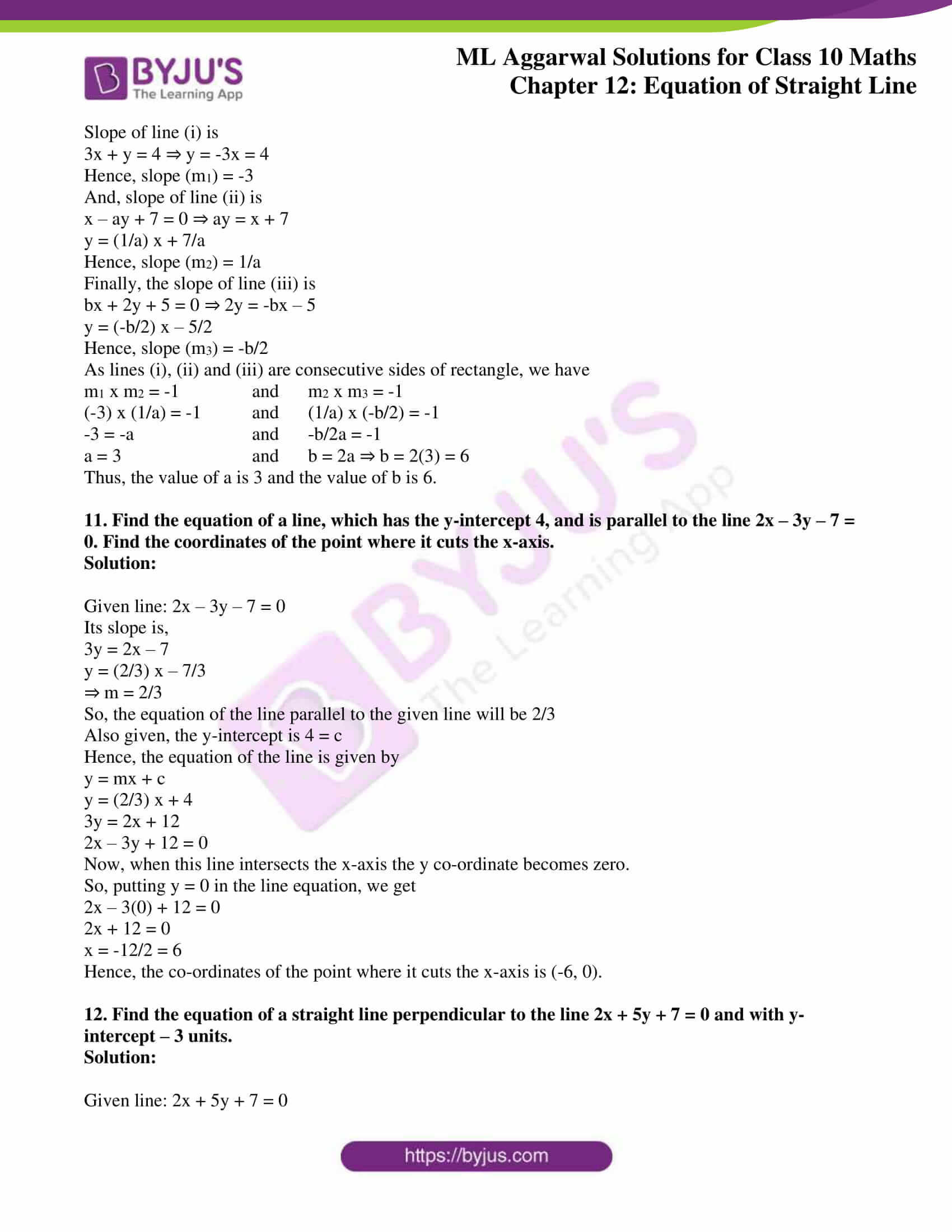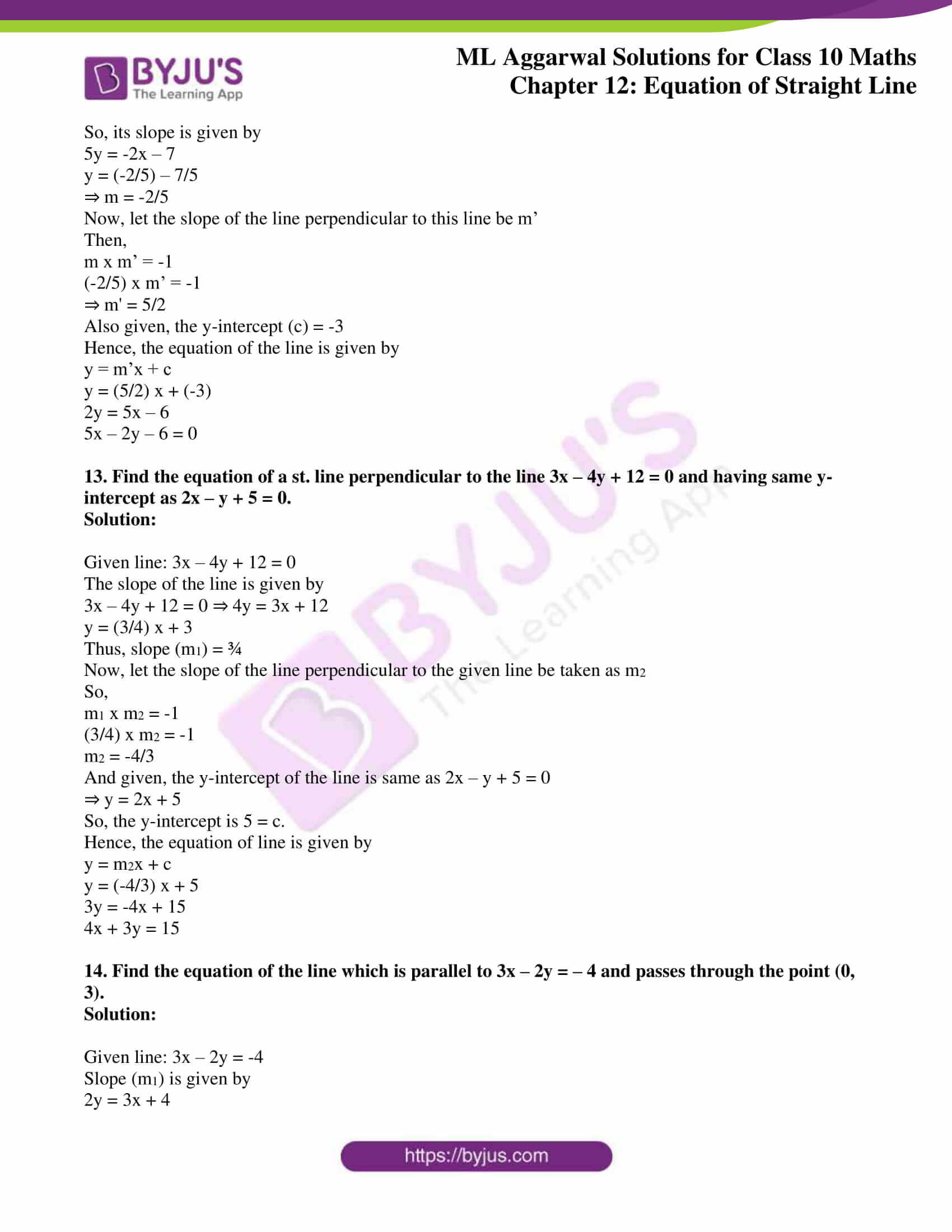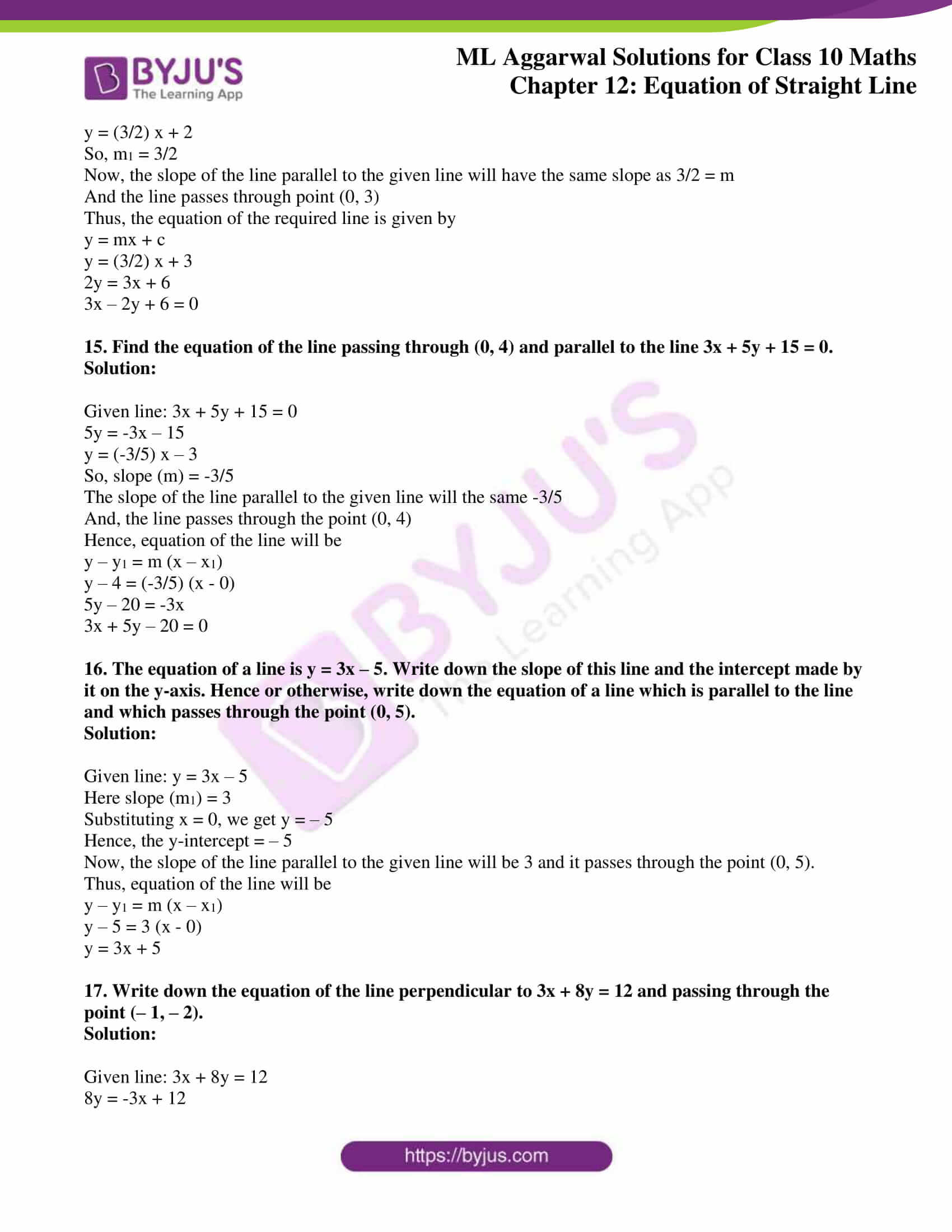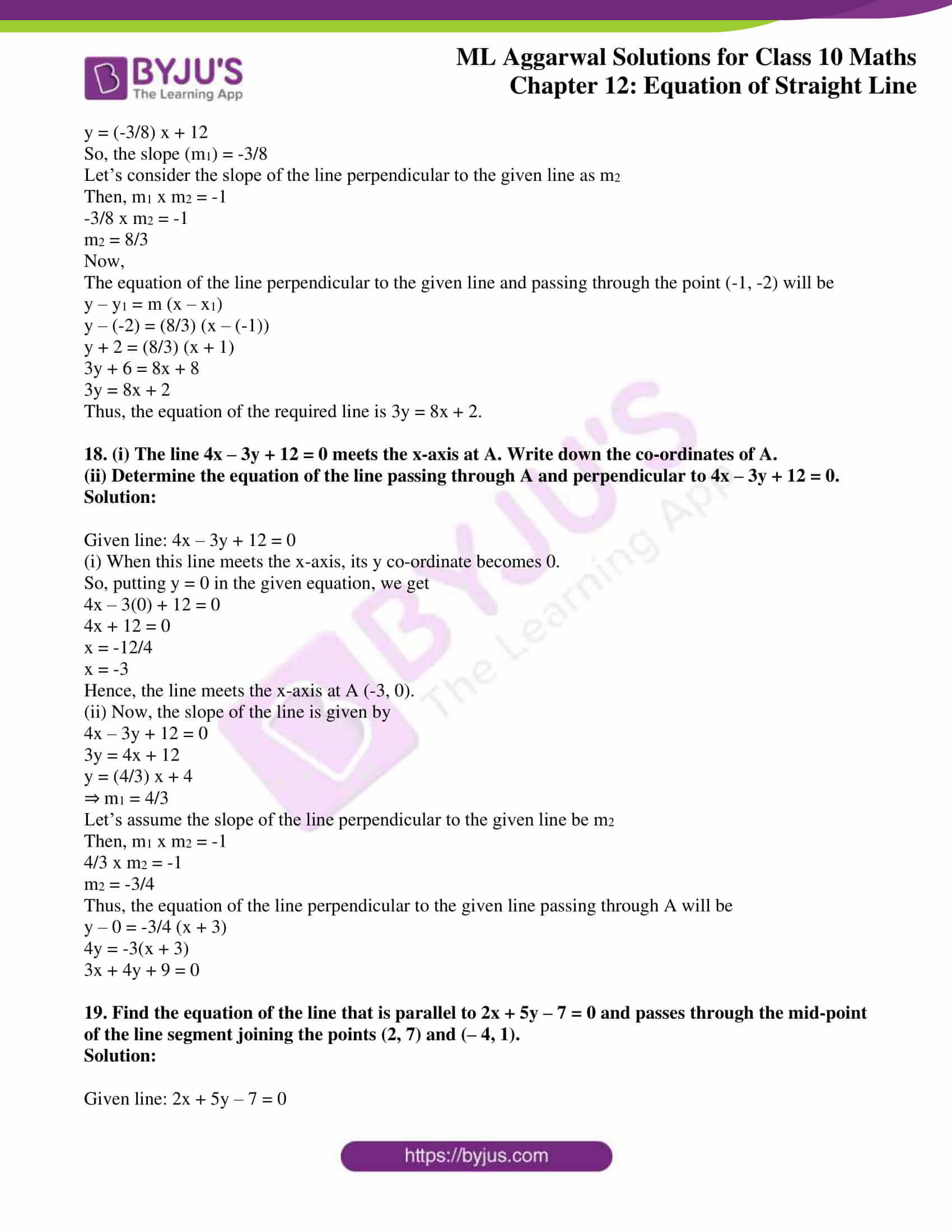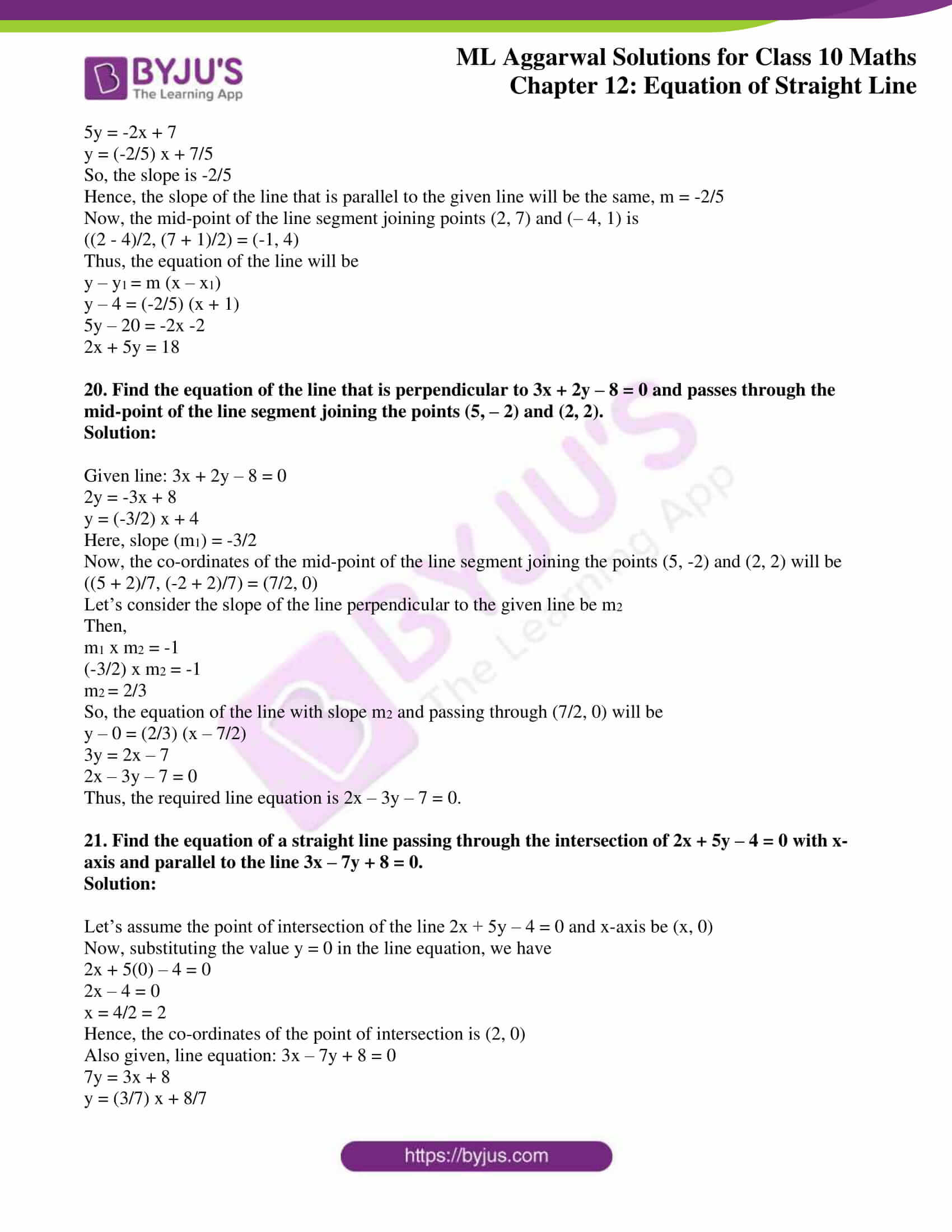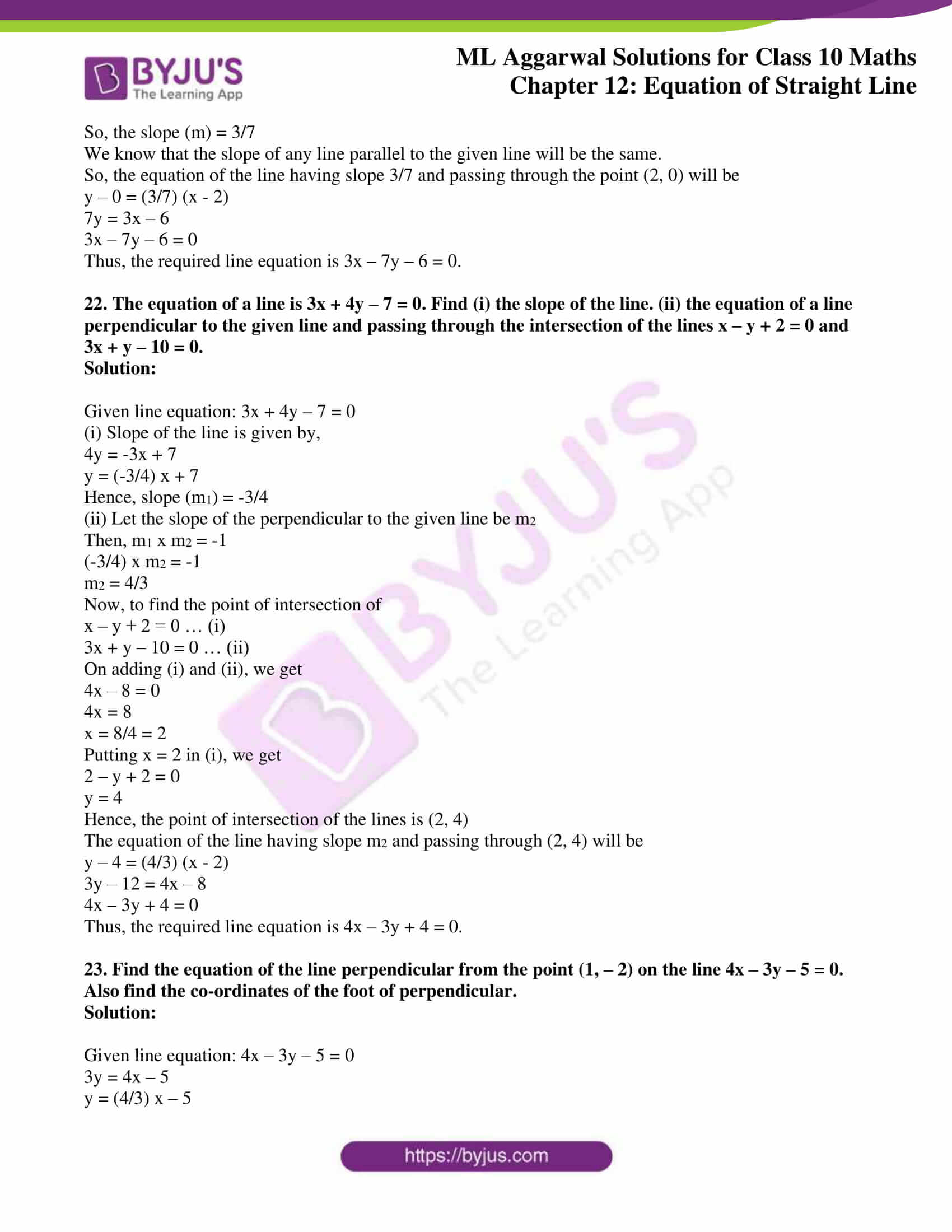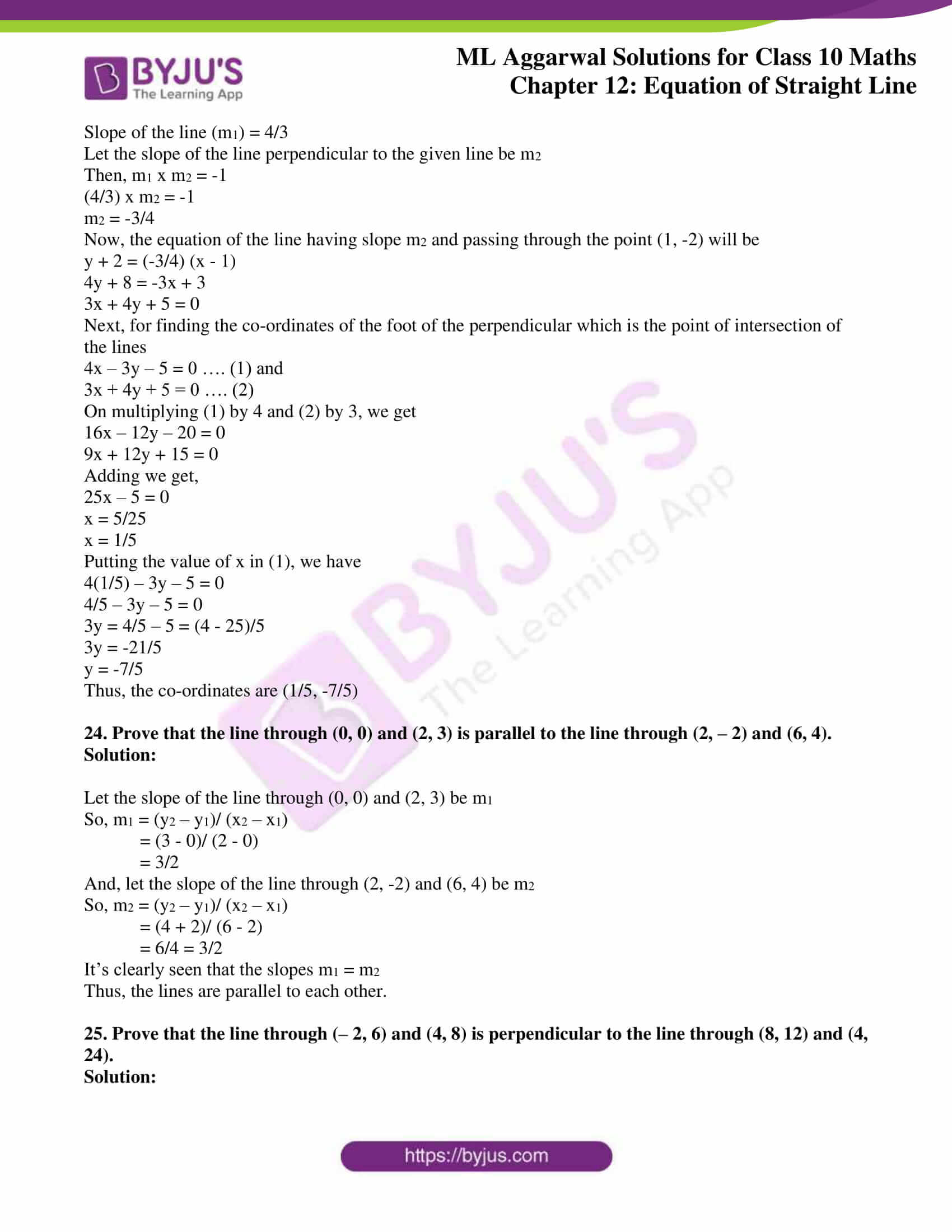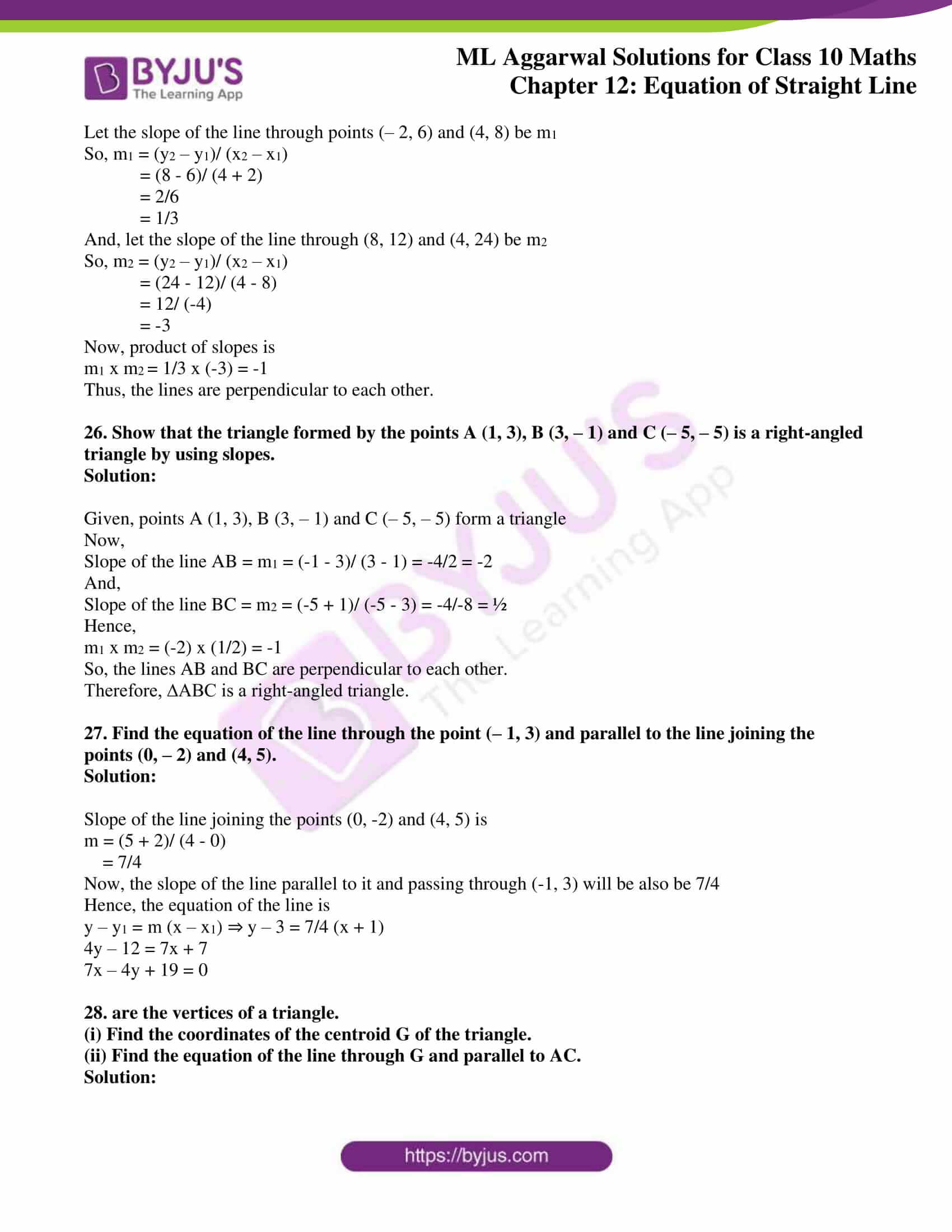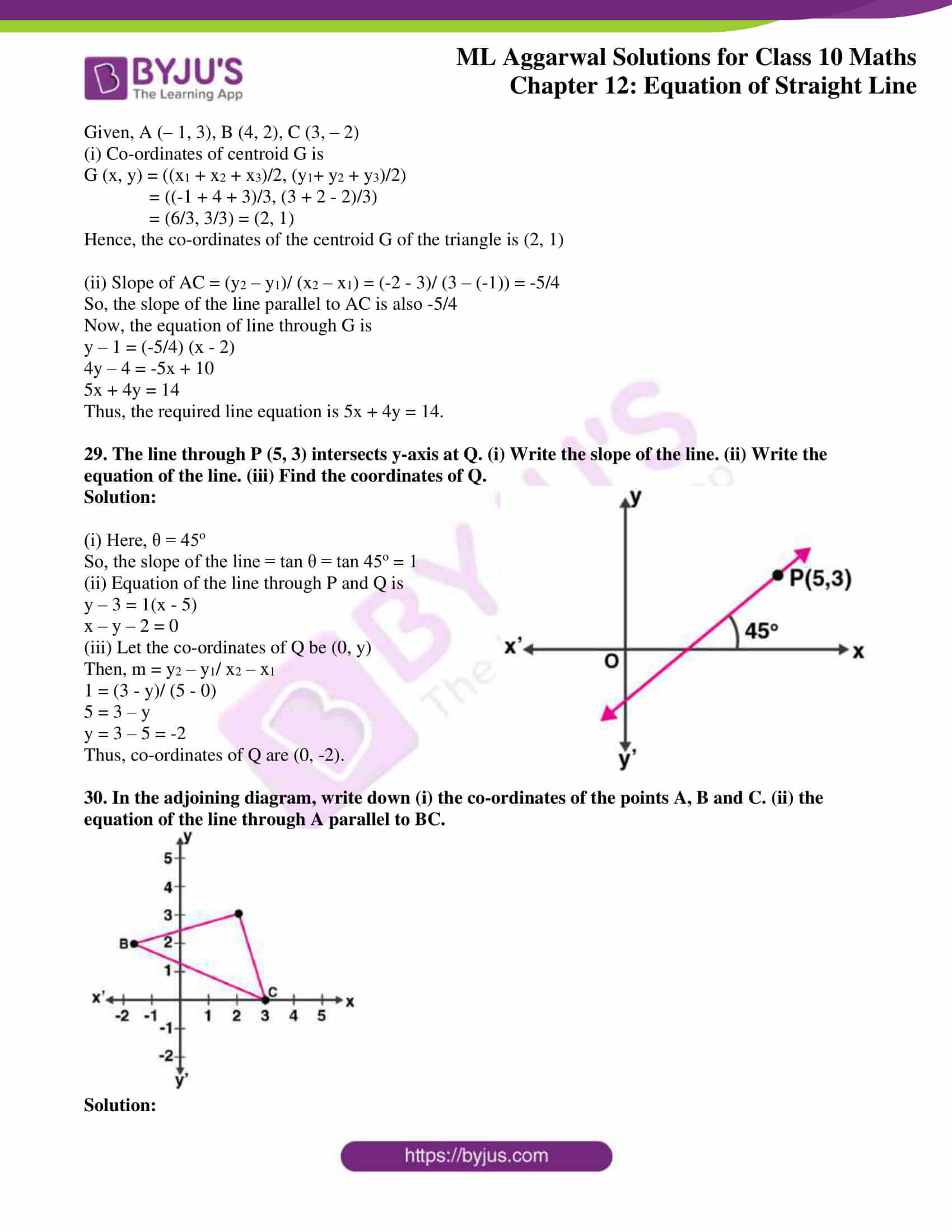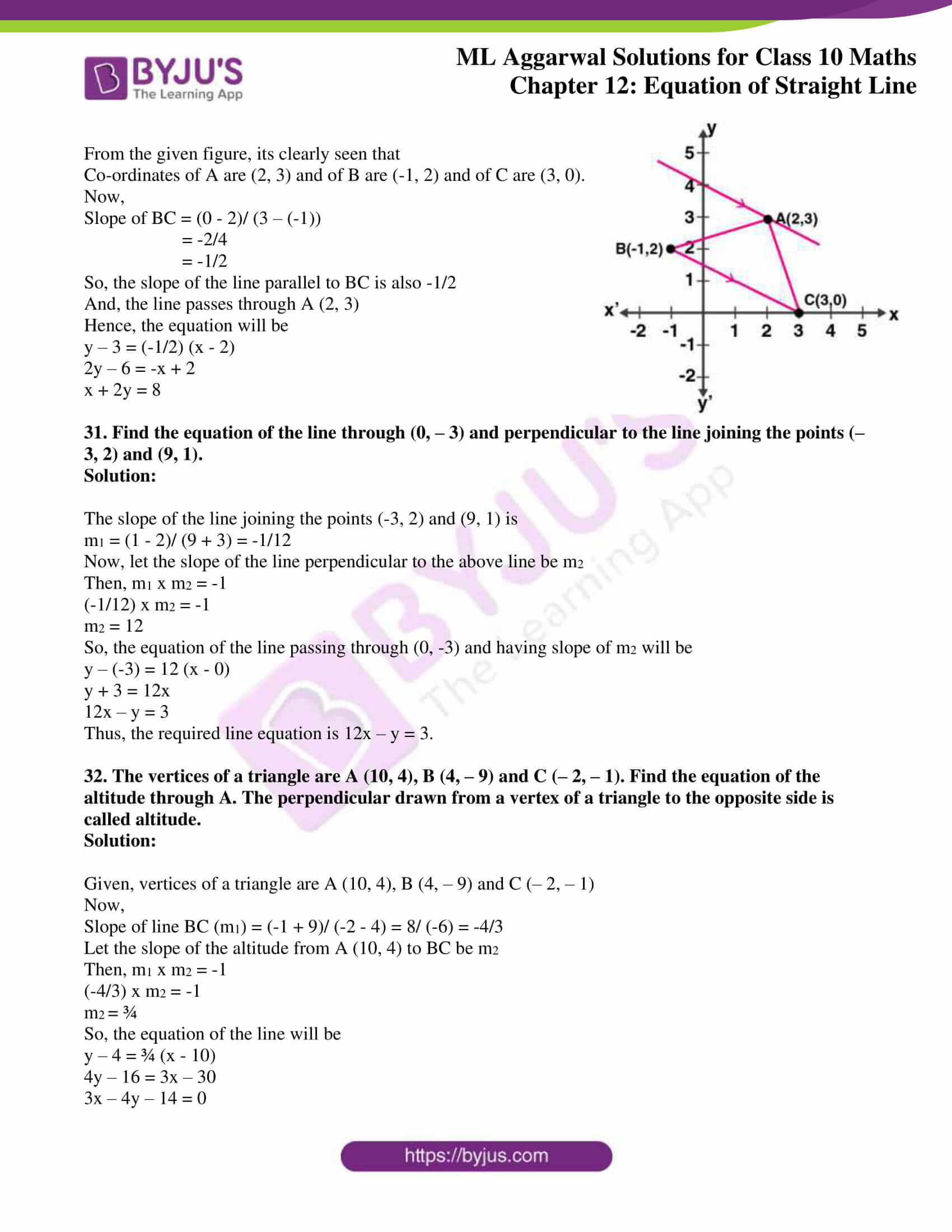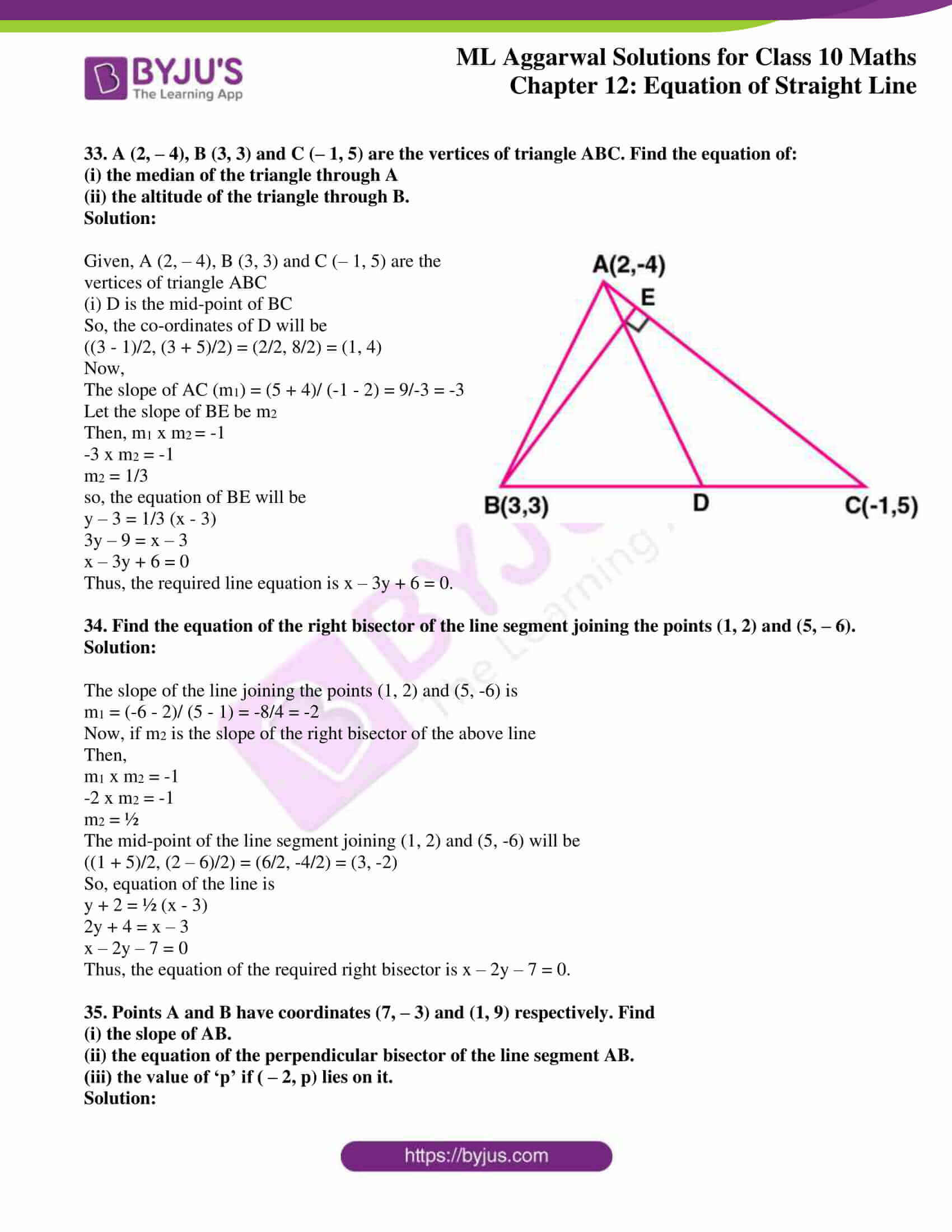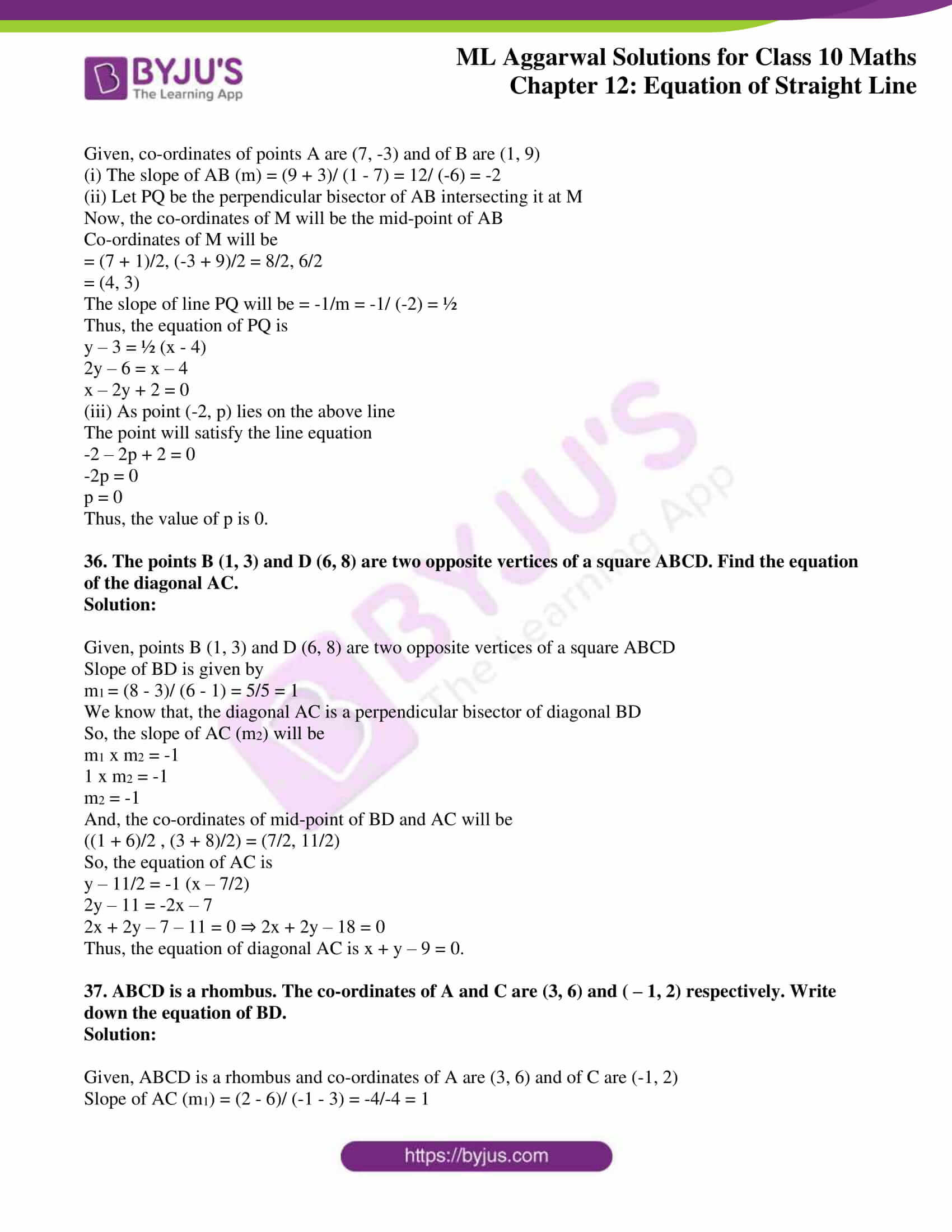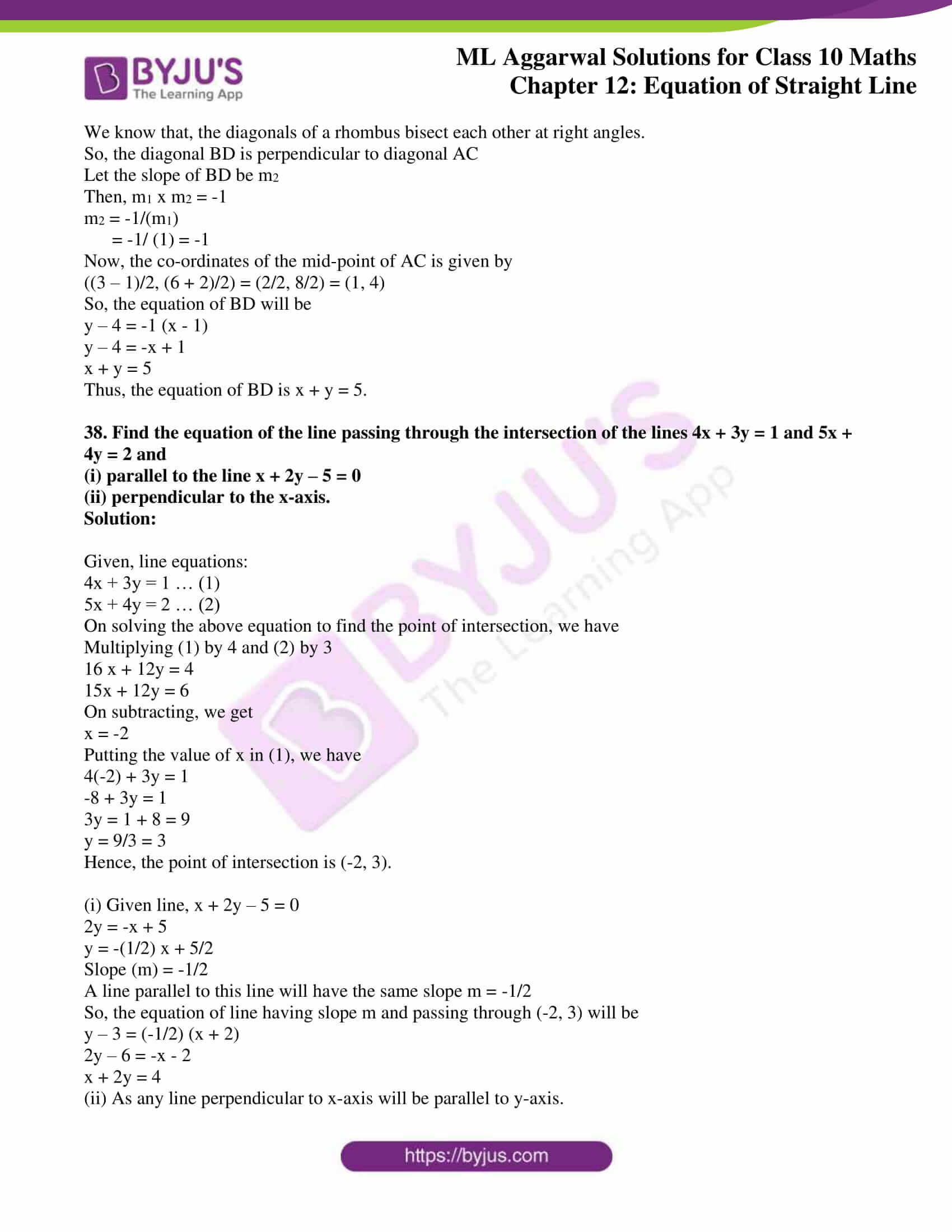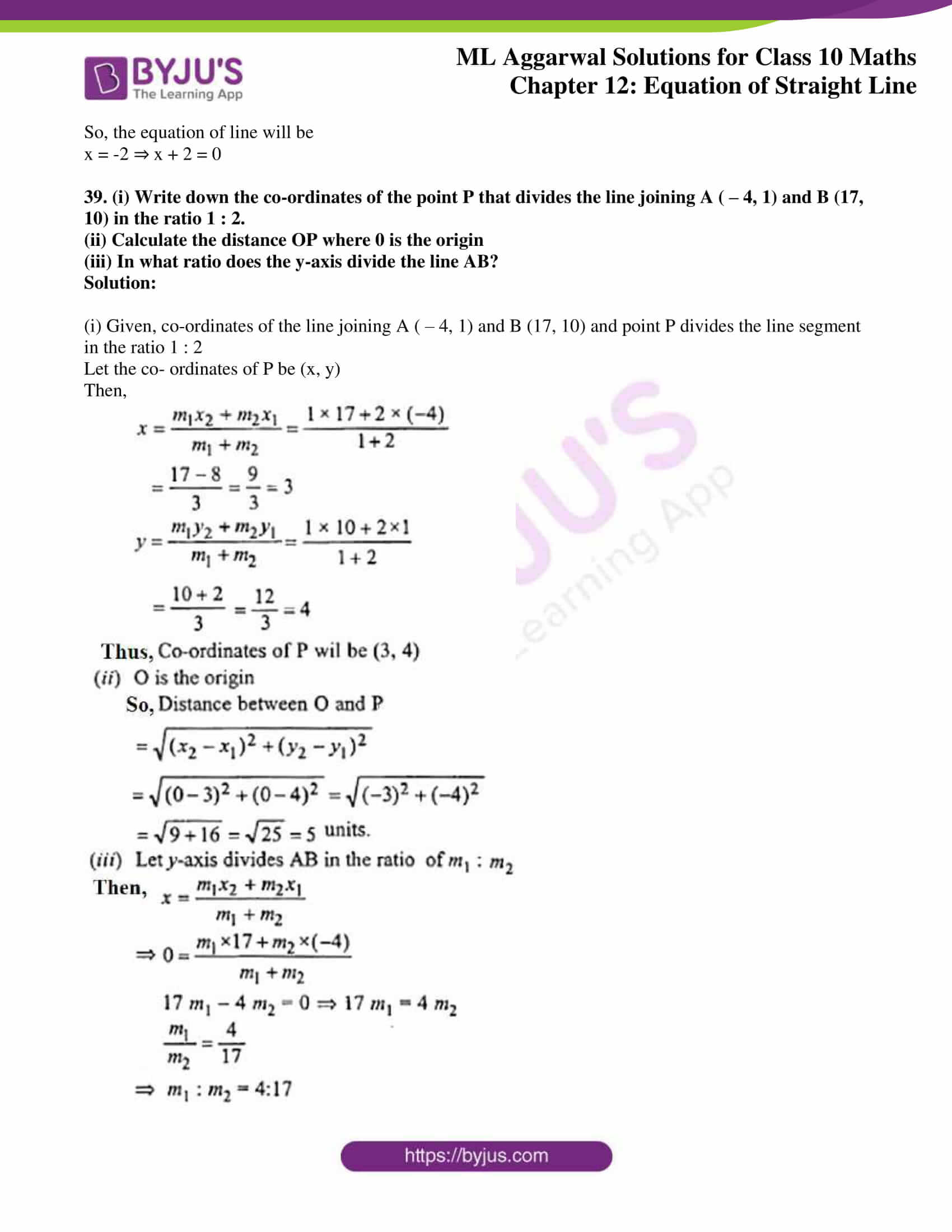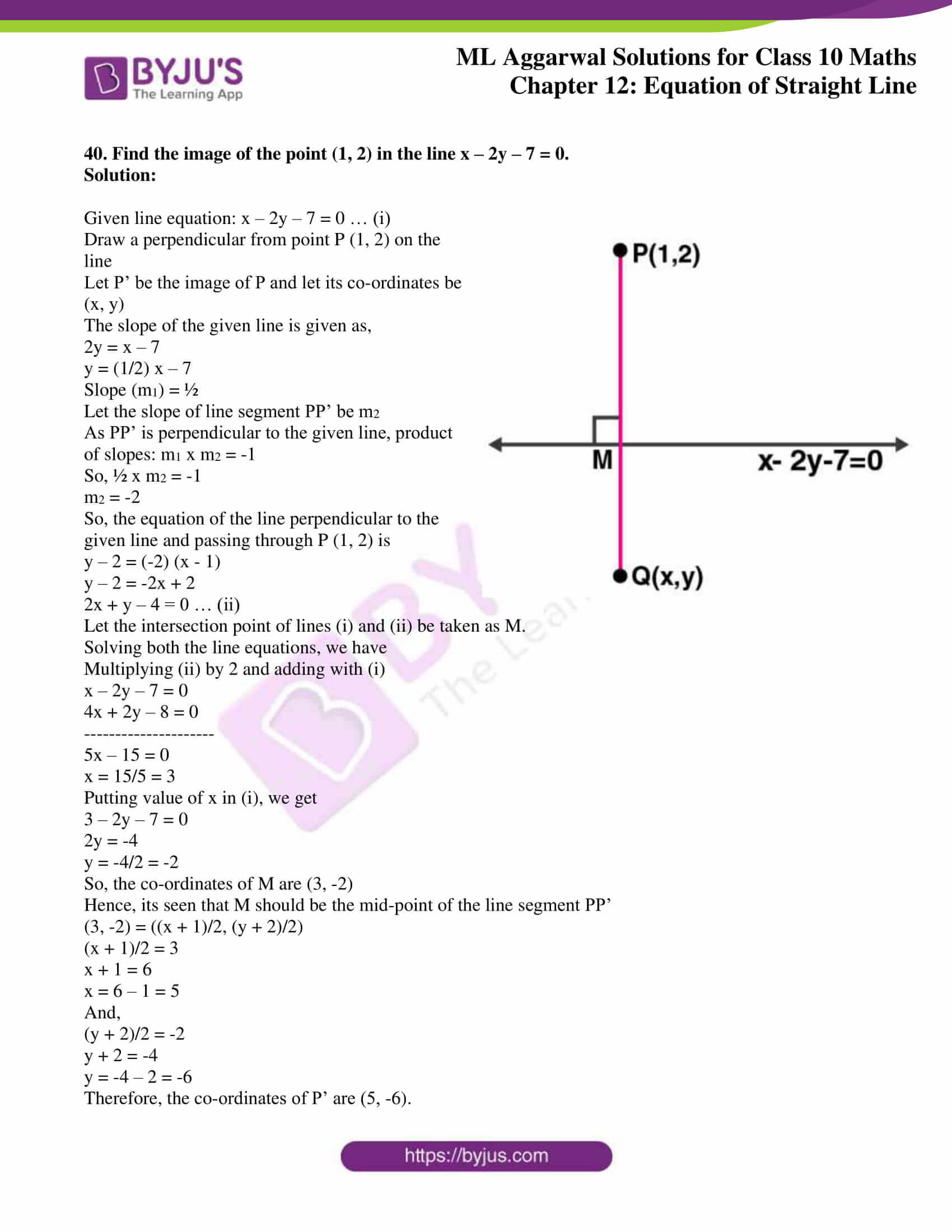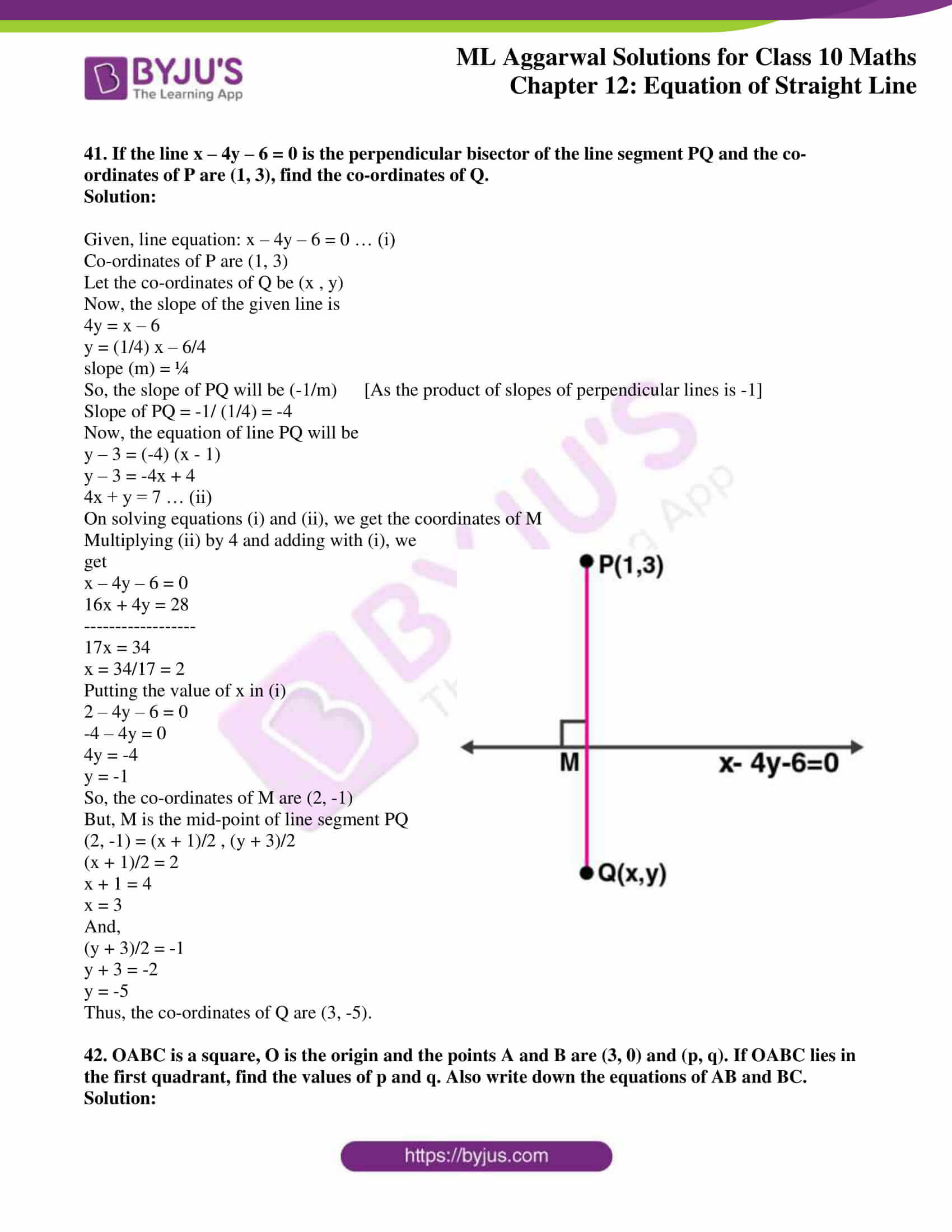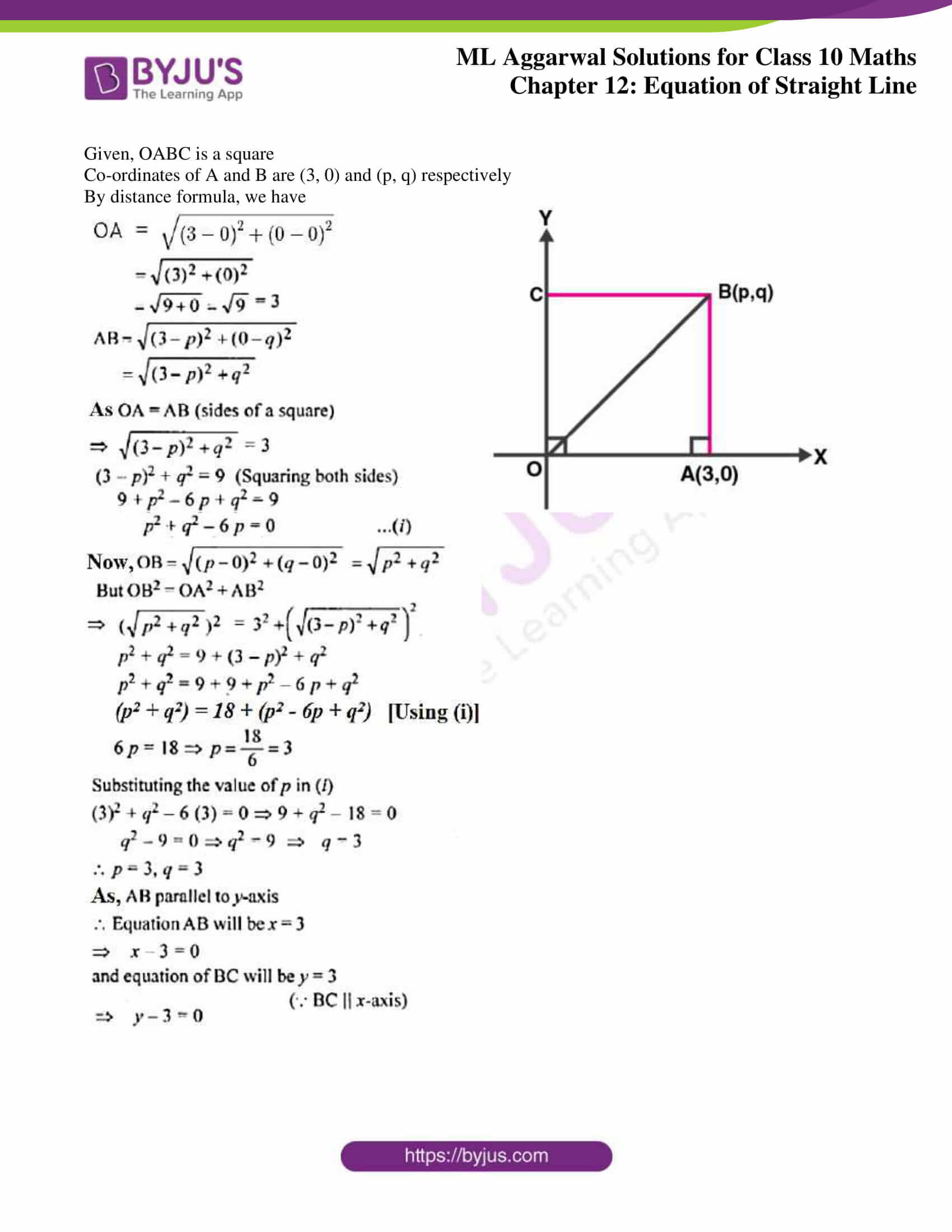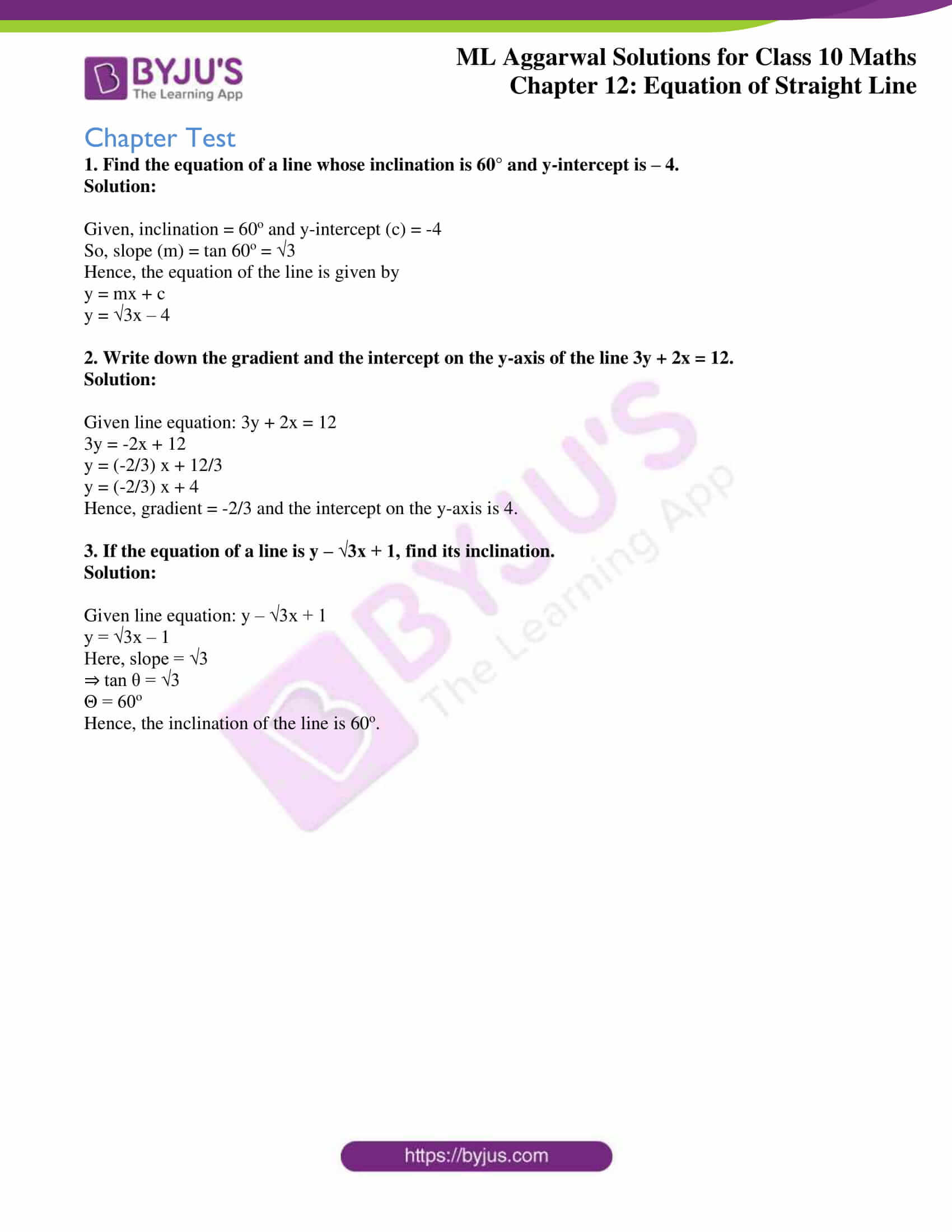## Access ML Aggarwal Solutions for Class 10 Maths Chapter 12 Equation of Straight Line

Exercise 12.1

1. Find the slope of a line whose inclination is
(i) 45°
(ii) 30°

Solution:

The slope of a line having inclination:

(i) 45o

Slope = tan 45o = 1

(ii) 30o

Slope = tan 30o = 1/√3

2. Find the inclination of a line whose gradient is
(i) 1
(ii) √3

(iii) 1/√3

Solution:

Given,

(i) tan θ = 1

⇒ θ = 45o

(ii) tan θ = 3

⇒ θ = 60o

(i) tan θ = 1/√3

⇒ θ = 30o

3. Find the equation of a straight line parallel to x-axis which is at a distance
(i) 2 units above it
(ii) 3 units below it.

Solution:

(i) A line which is parallel to x-axis is y = a

⇒ y = 2

Hence, the equation of line parallel to x-axis which is at a distance of 2 units above it is y – 2 = 0.

(ii) A line which is parallel to x-axis is y = a

⇒ y = -3

Hence, the equation of line parallel to x-axis which is at a distance of 3 units below it is y + 3 = 0.

4. Find the equation of a straight line parallel to y-axis which is at a distance of:
(i) 3 units to the right
(ii) 2 units to the left.

Solution:

A line which is parallel to y-axis is x = a

(i) Here, x = 3

Hence, the equation of line parallel to y-axis is at a distance of 3 units to the right is x – 3 = 0.

(ii) Here, x = -2

Hence, the equation of line parallel to y-axis at a distance of 2 units to the left is x + 2 = 0.

5. Find the equation of a straight line parallel to y-axis and passing through the point ( – 3, 5).

Solution:

The equation of the line parallel to y-axis passing through ( – 3, 5) to x = -3

⇒ x + 3 = 0

6. Find the equation of a line whose

(i) slope = 3, y-intercept = – 5

(ii) slope = -2/7, y-intercept = 3

(iii) gradient = √3, y-intercept = -4/3

(iv) inclination = 30°, y-intercept = 2

Solution:

Equation of a line whose slope and y-intercept is given by:

y = mx + c, where m is the slope and c is the y-intercept

(i) Given: slope = 3, y-intercept = – 5

⇒ y = 3x + (-5)

Hence, the equation of line is y = 3x – 5.

(ii) Given: slope = -2/7, y-intercept = 3

⇒ y = (-2/7)x + 3

y = (-2x + 21)/7

7y = -2x + 21

Hence, the equation of line is 2x + 7y – 21= 0.

(iii) Given: gradient = √3, y-intercept = -4/3

⇒ y = √3x + (-4/3)

y = (3√3x – 4)/3

3y = 3√3x – 4

Hence, the equation of line is 3√3x – 3y – 4 = 0.

(iv) Given: inclination = 30°, y-intercept = 2

Slope = tan 30o = 1/√3

⇒ y = (1/√3)x + 2

y = (x + 2√3)/ √3

√3y = x + 2√3

Hence, the equation of line is x – √3y + 2√3 = 0.

7. Find the slope and y-intercept of the following lines:

(i) x – 2y – 1 = 0

(ii) 4x – 5y – 9 = 0

(iii) 3x + 5y + 7 = 0

(iv) x/3 + y/4 = 1

(v) y – 3 = 0

(vi) x – 3 = 0

Solution:

We know that, equation of line whose slope and y-intercept is given by:

y = mx + c, where m is the slope and c is the y-intercept

Using the above and converting to this, we find

(i) x – 2y – 1 = 0

2y = x – 1

⇒ y = (½) x + (-½)

Hence, slope = ½ and y-intercept = – ½

(ii) 4x – 5y – 9 = 0

5y = 4x – 9

⇒ y = (4/5) x + (-9/5)

Hence, slope = 4/5 and y-intercept = -9/5

(iii) 3x + 5y + 7 = 0

5y = -3x – 7

⇒ y = (-3/5) x + (-7/5)

Hence, slope = -3/5 and y-intercept = -7/5

(iv) x/3 + y/4 = 1

(4x + 3y)/ 12 = 1

4x + 3y = 12

3y = -4x + 12

⇒ y = (-4/3) x + 4

Hence, slope = -4/3 and y-intercept = 4

(v) y – 3 = 0

y = 3

⇒ y = (0) x + 3

Hence, slope = 0 and y-intercept = 3

(vi) x – 3 = 0

Here, the slope cannot be defined as the line does not meet y-axis.

8. The equation of the line PQ is 3y – 3x + 7 = 0

(i) Write down the slope of the line PQ.

(ii) Calculate the angle that the line PQ makes with the positive direction of x-axis.

Solution:

Given, equation of line PQ is 3y – 3x + 7 = 0

Re-writing in form of y = mx + c, we have

3y = 3x – 7

⇒ y = x + (-7/3)

Here,

(i) Slope = 1

(ii) As tan θ = 1

θ = 45o

Hence, the angle which PQ makes with the x-axis is Q.

9. The given figure represents the line y = x + 1 and y = √3x – 1. Write down the angles which the lines make with the positive direction of the x-axis. Hence determine θ.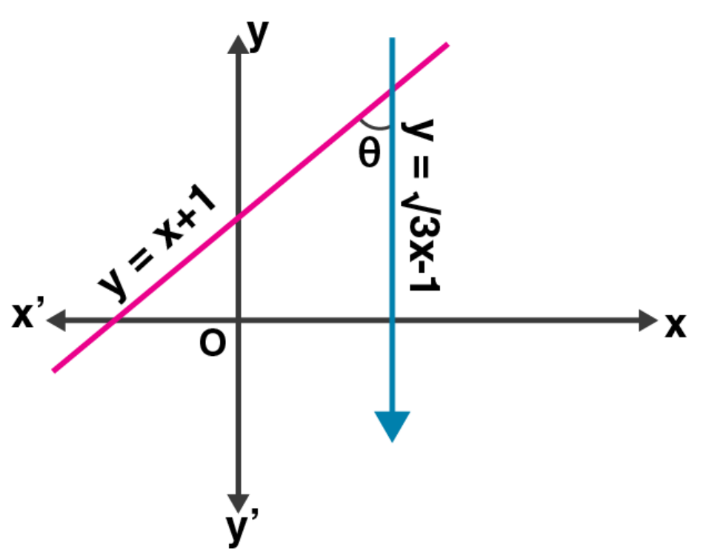Solution:

Given line equations, y = x + 1 and y = √3x – 1

On comparing with y = mx + c,

The slope of the line: y = x + 1 is 1 as m = 1

So, tan θ = 1 ⇒ θ = 45o

And,

The slope of the line: y = √3x – 1 is √3 as m = √3

So, tan θ = √3 ⇒ θ = 60o

Now, in triangle formed by the given two lines and x-axis

Ext. angle = Sum of interior opposite angle

60o = θ + 45o

θ = 60o – 45o

Thus, θ = 15o

10. Find the value of p, given that the line y/2 = x – p passes through the point (– 4, 4)

Solution:

Given, equation of line: y/2 = x – p

And, it passes through the point (-4, 4)

Hence, it satisfies the line equation

So,

4/2 = (-4) – p

2 = -4 – p

p = -4 – 2

Thus, p = -6

11. Given that (a, 2a) lies on the line y/2 = 3x – 6. Find the value of a.

Solution:

Given, equation of line: y/2 = 3x – 6

And, it passes through the point (a, 2a)

Hence, it satisfies the line equation

So,

2a/2 = 3(a) – 6

a = 3a – 6

2a = 6

Thus, a = 3

12. The graph of the equation y = mx + c passes through the points (1, 4) and (– 2, – 5). Determine the values of m and c.

Solution:

Given, equation of the line is y = mx + c

And, it passes through the points (1, 4)

So, the point will satisfy the line equation

⇒ 4 = m x 1 + c

4 = m + c

m + c = 4 … (i)

Also, the line passes through another point (-2, -5)

So,

5 = m (-2) + c

5 = -2 m + c

⇒ 2m – c = 5 …(ii)

Now, on adding (i) and (ii) we get

3m = 9

⇒ m = 3

Substituting the value of m in (i), we get

3 + c = 4

⇒ c = 4 – 3 = 1

Therefore, m = 3, c = 1

13. Find the equation of the line passing through the point (2, – 5) and making an intercept of – 3 on the y-axis.

Solution:

Given, a line equation passes through point (2, -5) and makes a y-intercept of -3

We know that,

The equation of line is y = mx + c, where m is the slope and c is the y-intercept

So, we have

y = mx – 3

Now, this line equation will satisfy the point (2, -5)

-5 = m (2) – 3

-5 = 2m – 3

2m = 3 – 5 = -2

⇒ m = -1

Hence, the equation of the line is y = -x + (-3) ⇒ x + y + 3 = 0

14. Find the equation of a straight line passing through (– 1, 2) and whose slope is 2/5.

Solution:

Given, the equation of straight line passes through (-1, 2) and having slope as 2/5

So, the equation of the line will be

y – y1 = m (x – x1)

Here, (x1, y1) is (-1, 2)

⇒ y – 2 = (2/5) [x – (-1)]

5 (y – 2) = 2 (x + 1)

5y – 10 = 2x + 2

Thus, the line equation is 2x – 5y + 12 = 0.

15. Find the equation of a straight line whose inclination is 60° and which passes through the point (0, – 3).

Solution:

Given,

Inclination of a straight line is 60o

So, the slope = tan 60o = √3 = m

And, the equation of line passes through the point (0, -3) = (x1, y1)

Hence, the equation of line is given by

y – y1 = m (x – x1)

y + 3 = √3 (x – 0)

y + 3 = √3x

√3x – y – 3 = 0

Thus, the line equation is √3x – y – 3 = 0.

16. Find the gradient of a line passing through the following pairs of points.
(i) (0, – 2), (3, 4)
(ii) (3, – 7), (– 1, 8)

Solution:

Gradient of a line (m) = y2 – y1 / x2 – x1

(i) (0, – 2), (3, 4)

m = (4 + 2)/(3 – 0) = 6/3 = 2

(ii) (3, – 7), (– 1, 8)

m = (8 + 7)/(-1 – 3) = 15/-4

17. The coordinates of two points E and F are (0, 4) and (3, 7) respectively. Find:
(ii) The equation of EF
(iii) The coordinates of the point where the line EF intersects the x-axis.

Solution:

Given, co-ordinates of points E and F are (0, 4) and (3, 7) respectively

m = y2 – y1 / x2 – x1 = (7 – 4)/(3 – 0) = 3/3

⇒ m = 1

(ii) Equation of line EF is given by,

y – y1 = m (x – x1)

y – 7 = 1 (x – 3)

y – 7 = x – 3

x – y + 7 – 3 = 0

Hence, the equation of line EF is x – y + 4 = 0

(iii) It’s seen that the co-ordinates of point of intersection of EF and the x-axis will be y = 0

So, substituting the value y = 0 in the above equation

x – y + 4 = 0

x – 0 + 4 = 0

x = -4

Hence, the co-ordinates are (-4, 0).

18. Find the intercepts made by the line 2x – 3y + 12 = 0 on the co-ordinate axis.

Solution:

Given line equation is 2x – 3y + 12 = 0

On putting y = 0, we will get the intercept made on x-axis

2x – 3y + 12 = 0

2x – 3 × 0 + 12 = 0,

2x – 0 + 2 = 0,

2x = -12

⇒ x = -6

Now, on putting x = 0, we get the intercepts made on y-axis

2x – 3y + 12 = 0

2 × 0 – 3y + 12 = 0

-3y = -12

⇒ y = 4

Hence, the x-intercept and y-intercept of the given line is -6 and 4 respectively.

19. Find the equation of the line passing through the points P (5, 1) and Q (1, – 1). Hence, show that the points P, Q and R (11, 4) are collinear.

Solution:

Given, two points P (5, 1) and G (1, -1)

Slope of the line (m) = y2 – y1/ x2 – x1

= -1 – 1/ 1 – 5

= -2/-4 = ½

So, the equation of the line is

y – y1 = m (x – x1)

y – 1 = ½ (x – 5)

2y – 2 = x – 5

x – 2y – 3 = 0

Now, if point R (11, 4) is collinear to points P and Q then, R (11, 4) should satisfy the line equation

On substituting, we have

11 – 2(4) – 3 = 11 – 8 – 3 = 0

As point R satisfies the line equation,

Hence, P, Q and R are collinear.

20. Find the value of ‘a’ for which the following points A (a, 3), B (2,1) and C (5, a) are collinear. Hence find the equation of the line.

Solution:

Given,

Points A (a, 3), B (2,1) and C (5, a) are collinear.

So, slope of AB = slope of BC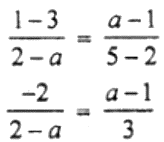⇒ -6 = (a – 1) (2 – a) [On cross multiplying]

-6 = 2a – 2 – a2 + a

-6 = 3a – a2 – 2

a2 – 3a – 4 = 0

a2 – 4a + a – 4 = 0

a (a – 4) + (a – 4) = 0

(a + 1) (a – 4) = 0

a = -1 or 4

As a = -1 doesn’t satisfy the equation

⇒ a = 4

Now,

Slope of BC = (a – 1)/(5 – 2) = (4 – 1)/3 = 3/3 = 1 = m

So, the equation of BC is

(y – 1) = 1 (x – 2)

y – 1 = x – 2

x – y = -1 + 2

Thus, the equation of BC is x – y = 1.

21. Use a graph paper for this question. The graph of a linear equation in x and y, passes through A (– 1, – 1) and B (2, 5). From your graph, find the values of h and k, if the line passes through (h, 4) and (½, k).

Solution:

Given,

Points (h, 4) and (1/2, k) lie on the line passing through A (-1, -1) and B (2, 5)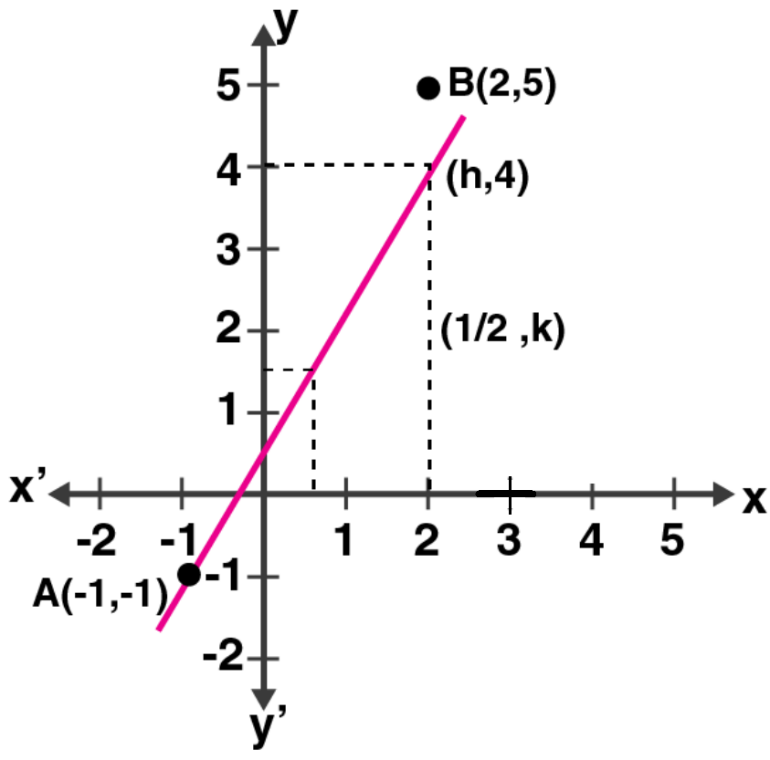From the graph, its clearly seen that

h = 3/2 and

k = 2

22. ABCD is a parallelogram where A (x, y), B (5, 8), C (4, 7) and D (2, – 4). Find
(i) the coordinates of A
(ii) the equation of the diagonal BD.

Solution:

(i) Given,

ABCD is a parallelogram where A (x, y), B (5, 8), C (4, 7) and D (2, -4)

O in the point of intersection of the diagonals of the parallelogram.

So, the co-ordinates of O = ([5 + 2]/2, [8 – 4]/2) = (3.5, 2)

Now, for the line AC we have

3.5 = (x + 4)/2 and 2 = (y + 7)/2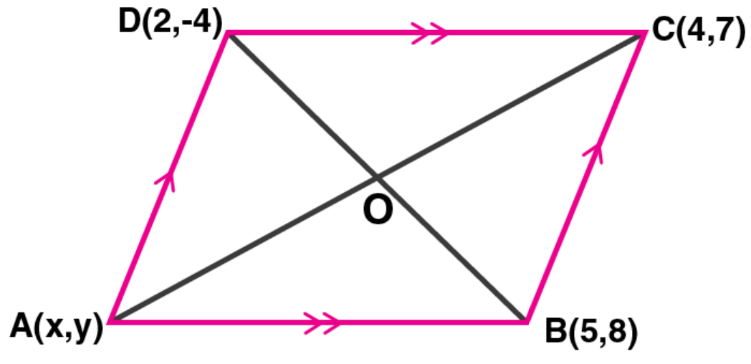7 = x + 4 and 4 = y + 7

x = 7 – 4 and y = 4 – 7

x = 3 and y = -3

Thus, the co-ordinates of A are (3, -3).

(ii) Equation of diagonal BD is given by

y – 8 = (-4 – 8)/(2 – 5) × (x – 5)

y – 8 = (-12/-3) × (x – 5)

y – 8 = 4 (x – 5)

y – 8 = 4x – 20

4x – y – 20 + 8 = 0

Hence, the equation of the diagonal is 4x – y – 12 = 0.

23. In ∆ABC, A (3, 5), B (7, 8) and C (1, – 10). Find the equation of the median through A.

Solution: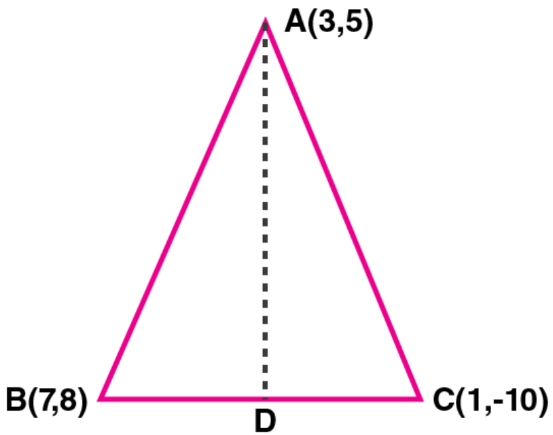Given,

∆ABC and their vertices A (3, 5), B (7, 8) and C (1, – 10).

So, D is mid-point of BC

Hence, the co-ordinates of D is ([7 + 1]/2, [8 – 10]/2) = (4, -1)

Now,

Slope of AD, m = y2 – y1/ x2 – x1

m = (5 + 1)/ (3 – 4) = 6/-1 = -6

Thus, the equation of AD is given by

y – y1 = m (x – x1)

y + 1 = -6 (x – 4)

y + 1 = -6x + 24

⇒ 6x + y – 23 = 0

24. Find the equation of a line passing through the point (– 2, 3) and having x-intercept 4 units.

Solution:

Given, point (-2, 3) and the x-intercept of the line passing through that point is 4 units.

So, the co-ordinates of the point where the line meets the x-axis is (4, 0)

Now, slope of the line passing through the points (-2, 3) and (4, 0)

m = y2 – y1/ x2 – x1

m = (0 – 3)/(4 + 2) = -3/6 = -1/2

Hence, the equation of the line will be

y – y1 = m (x – x1)

y – 0 = -½ (x – 4)

2y = -x + 4

⇒ x + 2y = 4

25. Find the equation of the line whose x-intercept is 6 and y-intercept is – 4.

Solution:

Given, x-intercept of a line is 6

So,

The line will pass through the point (6, 0)

Also given, the y -intercept of the line is -4 ⇒ c = -4

So, the line will pass through the point (0, -4)

Now,

Slope, m = m = y2 – y1/ x2 – x1

m = (-4 – 0)/(0 – 6) = -4/-6 = 2/3

Thus, the equation of the line is given by

y = mx + c

y = (2/3)x + (-4)

3y = 2x – 12

⇒ 2x – 3y – 12 = 0

26. Write down the equation of the line whose gradient is 3/2 and which passes through P where P divides the line segment joining A (– 2, 6) and B (3, – 4) in the ratio 2 : 3.

Solution:

Given, P divides the line segment joining the points A (-2, 6) and B (3, -4) in the ratio 2: 3

So, the co-ordinates of P will be

x = (m1x2 + m2x1)/(m1 + m2)

= (2×3 + 3×(-2))/ (2 + 3)

= (6 – 6)/5

= 0/5 = 0

y = (m1y2 + m2y1)/(m1 + m2)

= (2×(-4) + 3×(6))/ (2 + 3)

= (-8 + 18)/5 = 10/5

= 2

Hence, the co-ordinates of P are (0, 2)

Now, the slope (m) of the line passing through (0, 2) is 3/2

Thus, the equation will be

y – y1 = m (x – x1)

y – 2 = 3/2 (x – 0)

2y – 4 = 3x

⇒ 3x – 2y + 4 = 0

27. Find the equation of the line passing through the point (1, 4) and intersecting the line x – 2y – 11 = 0 on the y-axis.

Solution:

Given, line x – 2y – 11 = 0 passes through y-axis and point (1, 4)

So, putting x = 0 in the line equation we get the y-intercept

0 – 2y – 11 = 0

y = -11/2

The co-ordinates are (0, -11/2)

Now, the slope of the line joining the points (1, 4) and (0, -11/2) is given by

m = y2 – y1/ x2 – x1

= (-11/2 – 4)/ (0 – 1)

= 19/2

Thus, the line equation will be

y – y1 = m (x – x1)

y + 11/2 = 19/2 (x – 0)

2y + 11 = 19x

⇒ 19x – 2y – 11 = 0

28. Find the equation of the straight line containing the point (3, 2) and making positive equal intercepts on axes.

Solution:

Let the line containing the point P (3, 2) pass through x-axis at A (x, 0) and y-axis at B (0, y)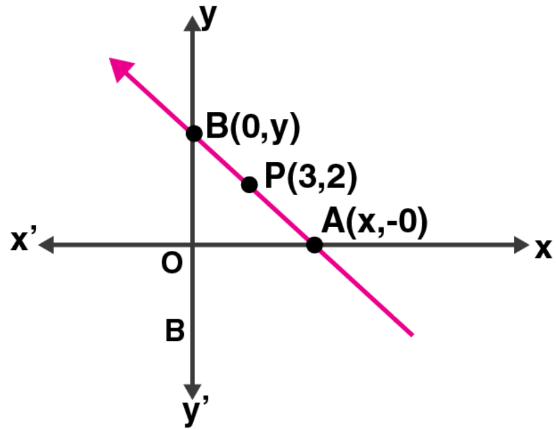Given, OA = OB

Thus, x = y

Now, the slope of the line (m) = y2 – y1/ x2 – x1

= 0 – y/ x – 0

= -x/x = -1

Hence, the equation of the line will be

y – y1 = m (x – x1)

y – 2 = -1 (x – 3)

y – 2 = -x + 3

⇒ x + y – 5 = 0

29. Three vertices of a parallelogram ABCD taken in order are A (3, 6), B (5, 10) and C (3, 2) find:
(i) the coordinates of the fourth vertex D.
(ii) length of diagonal BD.
(iii) equation of side AB of the parallelogram ABCD.

Solution:

Given, the three vertices of a parallelogram ABCD taken in order are A (3, 6), B (5, 10) and C (3, 2)

(i) We know that the diagonals of a parallelogram bisect each other.

Let (x, y) be the co-ordinates of D

Hence, we have

Mid-point of diagonal AC = ((3 + 3)/2, (6 + 2)/2) = (3, 4)

Mid-point of diagonal BD = ((5 + x)/2, (10 + y)/2)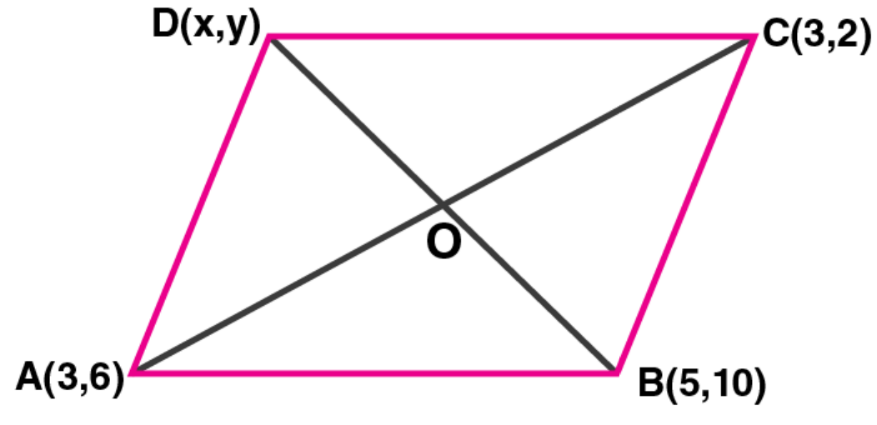And, these two should be the same

On equating we get,

(5 + x)/2 = 3 and (10 + y)/2 = 4

5 + x = 6 and 10 + y = 8

x = 1 and y = -2

Thus, the co-ordinates of D = (1, -2)

(ii) Length of diagonal BD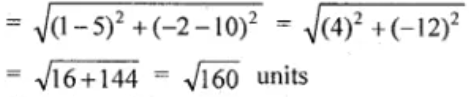(iii) Equation of the side joining A (3, 6) and D (1, -2) is given by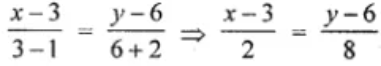4 (x – 3) = y – 6

4x – 12 = y – 6

4x – y = 6

Thus, the equation of the side joining A (3, 6) and D (1, -2) is 4x – y = 6.

30. A and B are two points on the x-axis and y-axis respectively. P (2, – 3) is the mid point of AB. Find the

(i) the co-ordinates of A and B.
(ii) the slope of the line AB.
(iii) the equation of the line AB.

Solution: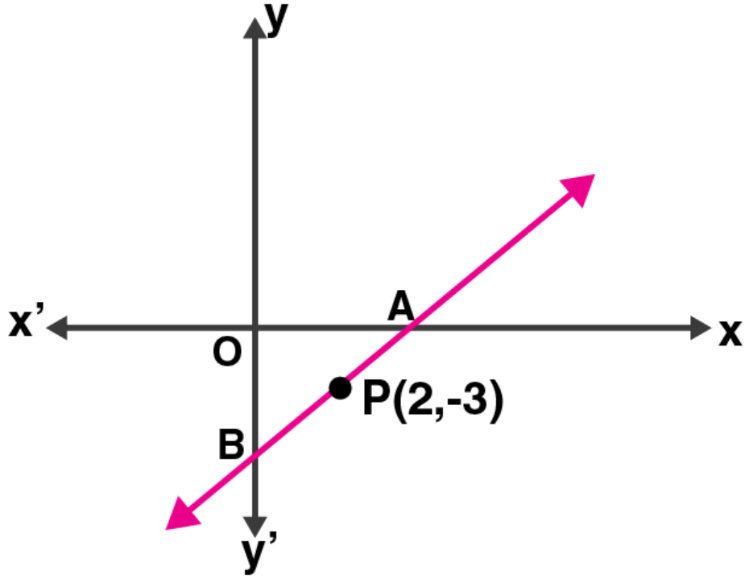Given, points A and B are on x-axis and y-axis respectively

Let co-ordinates of A be (x, 0) and of B be (0, y)

And P (2, -3) is the midpoint of AB

So, we have

2 = (x + 0)/2 and -3 = (0 + y)/2

x = 4 and y = -6

(i) Hence, the co-ordinates of A are (4, 0) and of B are (0, -6).

(ii) Slope of AB = y2 – y1/ x2 – x1

= (-6 – 0)/ (0 – 4)

= -6/-4 = 3/2 = m

(iii) Equation of AB will be

y – y1 = m (x – x1)

y – (-3) = 3/2 (x – 2) [As P lies on it]

y + 3 = 3/2 (x – 2)

2y + 6 = 3x – 6

3x – 2y – 12 = 0

31. Find the equations of the diagonals of a rectangle whose sides are x = – 1, x = 2, y = – 2 and y = 6.

Solution:

Given,

The equations of sides of a rectangle are

x1 = -1, x2 = 2, y1 = -2, y2 = 6.

These lines form a rectangle when they intersect at A, B, C, D respectively

Now,

The co-ordinates of A, B, C and D will be (-1, -2), (2, -2), (2, 6) and (-1, 6) respectively.

And, AC and BD are its diagonals

Slope of the diagonal AC

= y2 – y1/ x2 – x1

= (6 + 2)/ (2 + 1)

= 8/3 = m

So, the equation of AC will be

y – y1 = m (x – x1)

y + 2 = 8/3 (x + 1)

3y + 6 = 8x + 8

⇒ 8x – 3y + 2 = 0

32. Find the equation of a straight line passing through the origin and through the point of intersection of the lines 5x + 1y – 3 and 2x – 3y = 7

Solution:

Given line equations,

5x + 7y = 3 … (i)

2x – 3 y = 7 … (ii)

Now, performing multiplication of (i) by 3 and (ii) by 7, we get

15x + 21y = 9

14x – 21y = 49

29x = 58

x = 58/29 = 2

Substituting the value of x in (i), we get

5(2) + 7y = 3

10 + 7y = 3

7y = 3 – 10

y = -7/7 = -1

Hence, the point of intersection of lines is (2, -1)

Now, the slope of the line joining the points (2, -1) and (0, 0) will be

m = y2 – y1/ x2 – x1

= (0 + 1)/ (0 – 2)

= -1/2

Equation of the line is given by:

y – y1 = m (x – x1)

y – 0 = -1/2 (x – 0)

2y = -x

Thus, the required line equation is x + 2y = 0.

33. Point A (3, – 2) on reflection in the x-axis is mapped as A’ and point B on reflection in the y-axis is mapped onto B’ ( – 4, 3).
(i) Write down the co-ordinates of A’ and B.
(ii) Find the slope of the line A’B, hence find its inclination.

Solution:

Given,

A’ is the image of A (3, -2) on reflection in the x-axis.

(i) The co-ordinates of A’ will be (3, 2).

Again B’ (- 4, 3) in the image of A’, when reflected in the y-axis

Hence, the co-ordinates of B will be (4, 3)

(ii) Slope of the line joining, the points A’ (3, 2) and B (4, 3) will be

m = y2 – y1/ x2 – x1

= (2 – 3)/ (3 – 4)

= -1/-1 = 1

So, tan θ = 45o

Thus, the angle of inclination is 45o.

Exercise 12.2

1. State which one of the following is true: The straight lines y = 3x – 5 and 2y = 4x + 7 are

(i) parallel

(ii) perpendicular

(iii) neither parallel nor perpendicular.

Solution:

Given straight lines: y = 3x – 5 and 2y = 4x + 7 ⇒ y = 2x + 7/2

And, their slopes are 3 and 2

The product of slopes is 3 x 2 = 6.

Hence, as the slopes of both the lines are neither equal nor their product is -1 the given pair of straight lines are neither parallel nor perpendicular.

2. If 6x + 5y – 7 = 0 and 2px + 5y + 1 = 0 are parallel lines, find the value of p.

Solution:

For two lines to be parallel, their slopes must be same.

Given line equations,

6x + 5y – 7 = 0 and 2px + 5y + 1 = 0

In equation 6x + 5y – 7 = 0,

5y = -6x + 7

y = (-6/5) x + 7/5

So, the slope of the line (m1) = -6/5

Again, in equation 2px + 5y + 1 = 0

5y = -2px – 1

y = (-2p/5) x – 1/5

So, the slope of the line (m2) = -2p/5

For these two lines to be parallel

m1 = m2

-6/5 = -2p/5

p = (-6/5) x (-5/2)

Thus, p = 3

3. Lines 2x – by + 5 = 0 and ax + 3y = 2 are parallel. Find the relation connecting a and b.

Solution:

Given lines are: 2x – by + 5 = 0 and ax + 3y = 2

If two lines to be parallel then their slopes must be equal.

In equation 2x – by + 5 = 0,

by = 2x + 5

y = (2/b) x + 5/b

So, the slope of the line (m1) = 2/b

And in equation ax + 3y = 2,

3y = -ax + 2

y = (-a/3) x + 2/3

So, the slope of the line (m2) = (-a/3)

As the lines are parallel

m1 = m2

2/b = -a/3

6 = -ab

Hence, the relation connecting a and b is ab + 6 = 0

4. Given that the line y/2 = x – p and the line ax + 5 = 3y are parallel, find the value of a.

Solution:

Given,

Line equation: y/2 = x – p

⇒ y = 2x – 2p

Here, the slope of the line is 2.

And, another line equation: ax + 5 = 3y

⇒ 3y = ax + 5

y = (a/3) x + 5/3

Hence, the slope of the line is a/3

As the line are parallel, their slopes must be equal

⇒ 2 = a/3

a = 6

Thus, the value of a is 6.

5. If the lines y = 3x + 7 and 2y + px = 3 perpendicular to each other, find the value of p.

Solution:

If two lines are perpendicular, then the product of their slopes is -1

Now, slope of the line y = 3x + 7 is m1 = 3

And,

The slope of the line: 2y + px = 3

2y = -px + 3

y = (-p/2) x + 3

m2 = -p/2

As the lines as perpendicular,

⇒ m1 x m2 = -1

3 x (-p/2) = -1

p = 2/3

Thus, the value of p is 2/3.

6. If the straight lines kx – 5y + 4 = 0 and 4x – 2y + 5 = 0 are perpendicular to each other. Find the value of k.

Solution:

Given,

In equation, kx – 5y + 4 = 0

⇒ 5y = kx + 4

y = (k/5) x + 4/5

So, the slope (m1) = k/5

And, in equation 4x – 2y + 5 = 0

⇒ 2y = 4x + 5

y = 2x + 5/2

So, the slope (m2) = 2

As the lines are perpendicular to each other

⇒ m1 x m2 = -1

k/5 x 2 = -1

k = (-1 x 5)/2

Hence, the value of k = -5/2

7. If the lines 3x + by + 5 = 0 and ax – 5y + 7 = 0 are perpendicular to each other, find the relation connecting a and b.

Solution:

Given that the lines 3x + by + 5 = 0 and ax – 5y + 7 = 0 are perpendicular to each other

Then the product of their slopes must be -1.

Slope of line 3x + by + 5 = 0 is,

by = -3x – 5

y = (-3/b) – 5/b

So, slope (m1) = -3/b

And,

The slope of line ax – 5y + 7 = 0 is

5y = ax + 7

y = (a/5) x + 7/5

So, slope (m2) = a/5

As the lines are perpendicular, we have

m1 x m2 = -1

-3/b x a/5 = -1

-3a/5b = -1

-3a = – 5b

3a = 5b

Hence, the relation connecting a and b is 3a = 5b.

8. Is the line through (– 2, 3) and (4, 1) perpendicular to the line 3x = y + 1?
Does the line 3x = y + 1 bisect the join of (– 2, 3) and (4, 1).

Solution:

Slope of the line passing through the points (-2, 3) and (4, 1) is given by

m1 = y2 – y1/ x2 – x1

= (1 – 3)/ (4 + 2)

= -2/6

= -1/3

And, the slope of the line: 3x = y + 1

y = 3x -1

Slope (m2) = 3

Now,

m1 x m2 = -1/3 x 3 = -1

Thus, the lines are perpendicular to each other as the product of their slopes is -1.

Now,

Co-ordinates of the mid-point of the line joining the points (-2, 3) and (4, 1) is

([-2 + 4]/2, [3 + 1]/2) = (1, 2)

Now, if the line 3x = y + 1 passes through the mid-point then it will satisfy the equation

3(1) = (2) + 1

3 = 3

Hence, the line 3x = y + 1 bisects the line joining the points (– 2, 3) and (4, 1).

9. The line through A (– 2, 3) and B (4, b) is perpendicular to the line 2x – 4y = 5. Find the value of b.

Solution:

The slope of the line passing through A (-2, 3) and B (4, b) will be

m1 = (b – 3)/ (4 + 2) = (b – 3)/ 6

Now, the gradient of the given line 2x – 4y = 5 is

4y = 2x + 5

y = (2/4) x + 5/4

y = ½ x + 5/4

So, m2 = ½

As the line are perpendicular to each other, we have

m1 x m2 = -1

(b – 3)/ 6 × ½ = -1

(b – 3)/ 12 = -1

b – 3 = -12

b = -12 + 3 = -9

Hence, the value of b is -9.

10. If the lines 3x + y = 4, x – ay + 7 = 0 and bx + 2y + 5 = 0 form three consecutive sides of a rectangle, find the value of a and b.

Solution:

Given lines are:

3x + y = 4 … (i)

x – ay + 7 = 0 … (ii)

bx + 2y + 5 = 0 … (iii)

It’s said that these lines form three consecutive sides of a rectangle.

So,

Lines (i) and (ii) must be perpendicular

Also, lines (ii) and (iii) must be perpendicular

We know that, for two perpendicular lines the product of their slopes will be -1.

Now,

Slope of line (i) is

3x + y = 4 ⇒ y = -3x = 4

Hence, slope (m1) = -3

And, slope of line (ii) is

x – ay + 7 = 0 ⇒ ay = x + 7

y = (1/a) x + 7/a

Hence, slope (m2) = 1/a

Finally, the slope of line (iii) is

bx + 2y + 5 = 0 ⇒ 2y = -bx – 5

y = (-b/2) x – 5/2

Hence, slope (m3) = -b/2

As lines (i), (ii) and (iii) are consecutive sides of rectangle, we have

m1 x m2 = -1 and m2 x m3 = -1

(-3) x (1/a) = -1 and (1/a) x (-b/2) = -1

-3 = -a and -b/2a = -1

a = 3 and b = 2a ⇒ b = 2(3) = 6

Thus, the value of a is 3 and the value of b is 6.

11. Find the equation of a line, which has the y-intercept 4, and is parallel to the line 2x – 3y – 7 = 0. Find the coordinates of the point where it cuts the x-axis.

Solution:

Given line: 2x – 3y – 7 = 0

Its slope is,

3y = 2x – 7

y = (2/3) x – 7/3

⇒ m = 2/3

So, the equation of the line parallel to the given line will be 2/3

Also given, the y-intercept is 4 = c

Hence, the equation of the line is given by

y = mx + c

y = (2/3) x + 4

3y = 2x + 12

2x – 3y + 12 = 0

Now, when this line intersects the x-axis the y co-ordinate becomes zero.

So, putting y = 0 in the line equation, we get

2x – 3(0) + 12 = 0

2x + 12 = 0

x = -12/2 = 6

Hence, the co-ordinates of the point where it cuts the x-axis is (-6, 0).

12. Find the equation of a straight line perpendicular to the line 2x + 5y + 7 = 0 and with y-intercept – 3 units.

Solution:

Given line: 2x + 5y + 7 = 0

So, its slope is given by

5y = -2x – 7

y = (-2/5) – 7/5

⇒ m = -2/5

Now, let the slope of the line perpendicular to this line be m’

Then,

m x m’ = -1

(-2/5) x m’ = -1

⇒ m’ = 5/2

Also given, the y-intercept (c) = -3

Hence, the equation of the line is given by

y = m’x + c

y = (5/2) x + (-3)

2y = 5x – 6

5x – 2y – 6 = 0

13. Find the equation of a st. line perpendicular to the line 3x – 4y + 12 = 0 and having same y-intercept as 2x – y + 5 = 0.

Solution:

Given line: 3x – 4y + 12 = 0

The slope of the line is given by

3x – 4y + 12 = 0 ⇒ 4y = 3x + 12

y = (3/4) x + 3

Thus, slope (m1) = ¾

Now, let the slope of the line perpendicular to the given line be taken as m2

So,

m1 x m2 = -1

(3/4) x m2 = -1

m2 = -4/3

And given, the y-intercept of the line is same as 2x – y + 5 = 0

⇒ y = 2x + 5

So, the y-intercept is 5 = c.

Hence, the equation of line is given by

y = m2x + c

y = (-4/3) x + 5

3y = -4x + 15

4x + 3y = 15

14. Find the equation of the line which is parallel to 3x – 2y = – 4 and passes through the point (0, 3).

Solution:

Given line: 3x – 2y = -4

Slope (m1) is given by

2y = 3x + 4

y = (3/2) x + 2

So, m1 = 3/2

Now, the slope of the line parallel to the given line will have the same slope as 3/2 = m

And the line passes through point (0, 3)

Thus, the equation of the required line is given by

y = mx + c

y = (3/2) x + 3

2y = 3x + 6

3x – 2y + 6 = 0

15. Find the equation of the line passing through (0, 4) and parallel to the line 3x + 5y + 15 = 0.

Solution:

Given line: 3x + 5y + 15 = 0

5y = -3x – 15

y = (-3/5) x – 3

So, slope (m) = -3/5

The slope of the line parallel to the given line will the same -3/5

And, the line passes through the point (0, 4)

Hence, equation of the line will be

y – y1 = m (x – x1)

y – 4 = (-3/5) (x – 0)

5y – 20 = -3x

3x + 5y – 20 = 0

16. The equation of a line is y = 3x – 5. Write down the slope of this line and the intercept made by it on the y-axis. Hence or otherwise, write down the equation of a line which is parallel to the line and which passes through the point (0, 5).

Solution:

Given line: y = 3x – 5

Here slope (m1) = 3

Substituting x = 0, we get y = – 5

Hence, the y-intercept = – 5

Now, the slope of the line parallel to the given line will be 3 and it passes through the point (0, 5).

Thus, equation of the line will be

y – y1 = m (x – x1)

y – 5 = 3 (x – 0)

y = 3x + 5

17. Write down the equation of the line perpendicular to 3x + 8y = 12 and passing through the point (– 1, – 2).

Solution:

Given line: 3x + 8y = 12

8y = -3x + 12

y = (-3/8) x + 12

So, the slope (m1) = -3/8

Let’s consider the slope of the line perpendicular to the given line as m2

Then, m1 x m2 = -1

-3/8 x m2 = -1

m2 = 8/3

Now,

The equation of the line perpendicular to the given line and passing through the point (-1, -2) will be

y – y1 = m (x – x1)

y – (-2) = (8/3) (x – (-1))

y + 2 = (8/3) (x + 1)

3y + 6 = 8x + 8

3y = 8x + 2

Thus, the equation of the required line is 3y = 8x + 2.

18. (i) The line 4x – 3y + 12 = 0 meets the x-axis at A. Write down the co-ordinates of A.
(ii) Determine the equation of the line passing through A and perpendicular to 4x – 3y + 12 = 0.

Solution:

Given line: 4x – 3y + 12 = 0

(i) When this line meets the x-axis, its y co-ordinate becomes 0.

So, putting y = 0 in the given equation, we get

4x – 3(0) + 12 = 0

4x + 12 = 0

x = -12/4

x = -3

Hence, the line meets the x-axis at A (-3, 0).

(ii) Now, the slope of the line is given by

4x – 3y + 12 = 0

3y = 4x + 12

y = (4/3) x + 4

⇒ m1 = 4/3

Let’s assume the slope of the line perpendicular to the given line be m2

Then, m1 x m2 = -1

4/3 x m2 = -1

m2 = -3/4

Thus, the equation of the line perpendicular to the given line passing through A will be

y – 0 = -3/4 (x + 3)

4y = -3(x + 3)

3x + 4y + 9 = 0

19. Find the equation of the line that is parallel to 2x + 5y – 7 = 0 and passes through the mid-point of the line segment joining the points (2, 7) and (– 4, 1).

Solution:

Given line: 2x + 5y – 7 = 0

5y = -2x + 7

y = (-2/5) x + 7/5

So, the slope is -2/5

Hence, the slope of the line that is parallel to the given line will be the same, m = -2/5

Now, the mid-point of the line segment joining points (2, 7) and (– 4, 1) is

((2 – 4)/2, (7 + 1)/2) = (-1, 4)

Thus, the equation of the line will be

y – y1 = m (x – x1)

y – 4 = (-2/5) (x + 1)

5y – 20 = -2x -2

2x + 5y = 18

20. Find the equation of the line that is perpendicular to 3x + 2y – 8 = 0 and passes through the mid-point of the line segment joining the points (5, – 2) and (2, 2).

Solution:

Given line: 3x + 2y – 8 = 0

2y = -3x + 8

y = (-3/2) x + 4

Here, slope (m1) = -3/2

Now, the co-ordinates of the mid-point of the line segment joining the points (5, -2) and (2, 2) will be

((5 + 2)/7, (-2 + 2)/7) = (7/2, 0)

Let’s consider the slope of the line perpendicular to the given line be m2

Then,

m1 x m2 = -1

(-3/2) x m2 = -1

m2 = 2/3

So, the equation of the line with slope m2 and passing through (7/2, 0) will be

y – 0 = (2/3) (x – 7/2)

3y = 2x – 7

2x – 3y – 7 = 0

Thus, the required line equation is 2x – 3y – 7 = 0.

21. Find the equation of a straight line passing through the intersection of 2x + 5y – 4 = 0 with x-axis and parallel to the line 3x – 7y + 8 = 0.

Solution:

Let’s assume the point of intersection of the line 2x + 5y – 4 = 0 and x-axis be (x, 0)

Now, substituting the value y = 0 in the line equation, we have

2x + 5(0) – 4 = 0

2x – 4 = 0

x = 4/2 = 2

Hence, the co-ordinates of the point of intersection is (2, 0)

Also given, line equation: 3x – 7y + 8 = 0

7y = 3x + 8

y = (3/7) x + 8/7

So, the slope (m) = 3/7

We know that the slope of any line parallel to the given line will be the same.

So, the equation of the line having slope 3/7 and passing through the point (2, 0) will be

y – 0 = (3/7) (x – 2)

7y = 3x – 6

3x – 7y – 6 = 0

Thus, the required line equation is 3x – 7y – 6 = 0.

22. The equation of a line is 3x + 4y – 7 = 0. Find (i) the slope of the line. (ii) the equation of a line perpendicular to the given line and passing through the intersection of the lines x – y + 2 = 0 and 3x + y – 10 = 0.

Solution:

Given line equation: 3x + 4y – 7 = 0

(i) Slope of the line is given by,

4y = -3x + 7

y = (-3/4) x + 7

Hence, slope (m1) = -3/4

(ii) Let the slope of the perpendicular to the given line be m2

Then, m1 x m2 = -1

(-3/4) x m2 = -1

m2 = 4/3

Now, to find the point of intersection of

x – y + 2 = 0 … (i)

3x + y – 10 = 0 … (ii)

On adding (i) and (ii), we get

4x – 8 = 0

4x = 8

x = 8/4 = 2

Putting x = 2 in (i), we get

2 – y + 2 = 0

y = 4

Hence, the point of intersection of the lines is (2, 4)

The equation of the line having slope m2 and passing through (2, 4) will be

y – 4 = (4/3) (x – 2)

3y – 12 = 4x – 8

4x – 3y + 4 = 0

Thus, the required line equation is 4x – 3y + 4 = 0.

23. Find the equation of the line perpendicular from the point (1, – 2) on the line 4x – 3y – 5 = 0. Also find the co-ordinates of the foot of perpendicular.

Solution:

Given line equation: 4x – 3y – 5 = 0

3y = 4x – 5

y = (4/3) x – 5

Slope of the line (m1) = 4/3

Let the slope of the line perpendicular to the given line be m2

Then, m1 x m2 = -1

(4/3) x m2 = -1

m2 = -3/4

Now, the equation of the line having slope m2 and passing through the point (1, -2) will be

y + 2 = (-3/4) (x – 1)

4y + 8 = -3x + 3

3x + 4y + 5 = 0

Next, for finding the co-ordinates of the foot of the perpendicular which is the point of intersection of the lines

4x – 3y – 5 = 0 …. (1) and

3x + 4y + 5 = 0 …. (2)

On multiplying (1) by 4 and (2) by 3, we get

16x – 12y – 20 = 0

9x + 12y + 15 = 0

25x – 5 = 0

x = 5/25

x = 1/5

Putting the value of x in (1), we have

4(1/5) – 3y – 5 = 0

4/5 – 3y – 5 = 0

3y = 4/5 – 5 = (4 – 25)/5

3y = -21/5

y = -7/5

Thus, the co-ordinates are (1/5, -7/5)

24. Prove that the line through (0, 0) and (2, 3) is parallel to the line through (2, – 2) and (6, 4).

Solution:

Let the slope of the line through (0, 0) and (2, 3) be m1

So, m1 = (y2 – y1)/ (x2 – x1)

= (3 – 0)/ (2 – 0)

= 3/2

And, let the slope of the line through (2, -2) and (6, 4) be m2

So, m2 = (y2 – y1)/ (x2 – x1)

= (4 + 2)/ (6 – 2)

= 6/4 = 3/2

It’s clearly seen that the slopes m1 = m2

Thus, the lines are parallel to each other.

25. Prove that the line through (– 2, 6) and (4, 8) is perpendicular to the line through (8, 12) and (4, 24).

Solution:

Let the slope of the line through points (– 2, 6) and (4, 8) be m1

So, m1 = (y2 – y1)/ (x2 – x1)

= (8 – 6)/ (4 + 2)

= 2/6

= 1/3

And, let the slope of the line through (8, 12) and (4, 24) be m2

So, m2 = (y2 – y1)/ (x2 – x1)

= (24 – 12)/ (4 – 8)

= 12/ (-4)

= -3

Now, product of slopes is

m1 x m2 = 1/3 x (-3) = -1

Thus, the lines are perpendicular to each other.

26. Show that the triangle formed by the points A (1, 3), B (3, – 1) and C (– 5, – 5) is a right-angled triangle by using slopes.

Solution:

Given, points A (1, 3), B (3, – 1) and C (– 5, – 5) form a triangle

Now,

Slope of the line AB = m1 = (-1 – 3)/ (3 – 1) = -4/2 = -2

And,

Slope of the line BC = m2 = (-5 + 1)/ (-5 – 3) = -4/-8 = ½

Hence,

m1 x m2 = (-2) x (1/2) = -1

So, the lines AB and BC are perpendicular to each other.

Therefore, ∆ABC is a right-angled triangle.

27. Find the equation of the line through the point (– 1, 3) and parallel to the line joining the points (0, – 2) and (4, 5).

Solution:

Slope of the line joining the points (0, -2) and (4, 5) is

m = (5 + 2)/ (4 – 0)

= 7/4

Now, the slope of the line parallel to it and passing through (-1, 3) will be also be 7/4

Hence, the equation of the line is

y – y1 = m (x – x1) ⇒ y – 3 = 7/4 (x + 1)

4y – 12 = 7x + 7

7x – 4y + 19 = 0

28. are the vertices of a triangle.

(i) Find the coordinates of the centroid G of the triangle.

(ii) Find the equation of the line through G and parallel to AC.

Solution:

Given, A (– 1, 3), B (4, 2), C (3, – 2)

(i) Co-ordinates of centroid G is

G (x, y) = ((x1 + x2 + x3)/2, (y1+ y2 + y3)/2)

= ((-1 + 4 + 3)/3, (3 + 2 – 2)/3)

= (6/3, 3/3) = (2, 1)

Hence, the co-ordinates of the centroid G of the triangle is (2, 1)

(ii) Slope of AC = (y2 – y1)/ (x2 – x1) = (-2 – 3)/ (3 – (-1)) = -5/4

So, the slope of the line parallel to AC is also -5/4

Now, the equation of line through G is

y – 1 = (-5/4) (x – 2)

4y – 4 = -5x + 10

5x + 4y = 14

Thus, the required line equation is 5x + 4y = 14.

29. The line through P (5, 3) intersects y-axis at Q. (i) Write the slope of the line. (ii) Write the equation of the line. (iii) Find the coordinates of Q.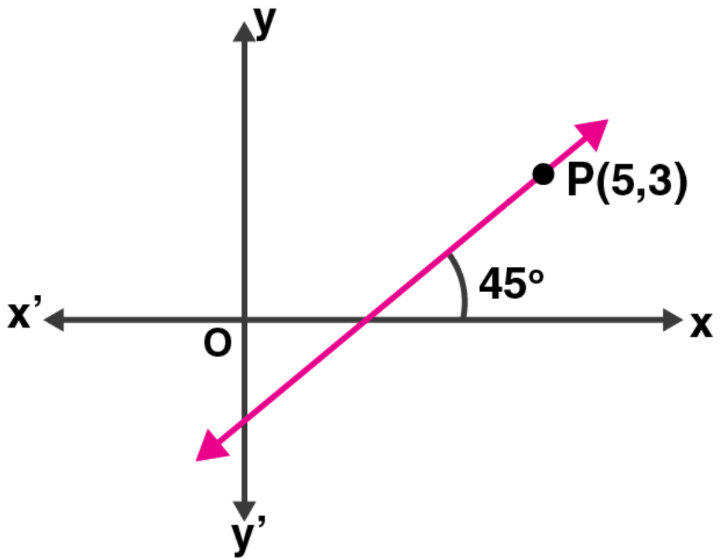Solution:

(i) Here, θ = 45o

So, the slope of the line = tan θ = tan 45o = 1

(ii) Equation of the line through P and Q is

y – 3 = 1(x – 5)

x – y – 2 = 0

(iii) Let the co-ordinates of Q be (0, y)

Then, m = y2 – y1/ x2 – x1

1 = (3 – y)/ (5 – 0)

5 = 3 – y

y = 3 – 5 = -2

Thus, co-ordinates of Q are (0, -2).

30. In the adjoining diagram, write down (i) the co-ordinates of the points A, B and C. (ii) the equation of the line through A parallel to BC.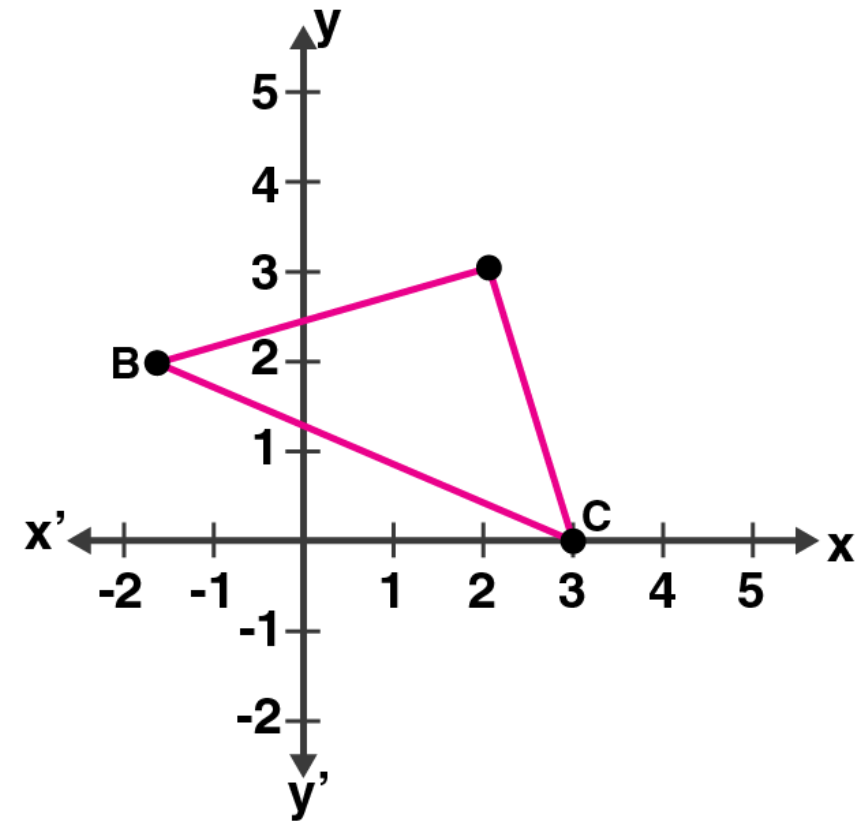Solution: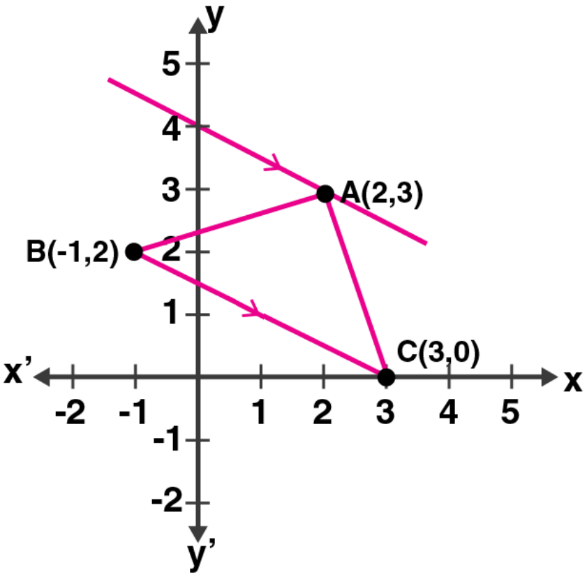From the given figure, its clearly seen that

Co-ordinates of A are (2, 3) and of B are (-1, 2) and of C are (3, 0).

Now,

Slope of BC = (0 – 2)/ (3 – (-1))

= -2/4

= -1/2

So, the slope of the line parallel to BC is also -1/2

And, the line passes through A (2, 3)

Hence, the equation will be

y – 3 = (-1/2) (x – 2)

2y – 6 = -x + 2

x + 2y = 8

31. Find the equation of the line through (0, – 3) and perpendicular to the line joining the points (– 3, 2) and (9, 1).

Solution:

The slope of the line joining the points (-3, 2) and (9, 1) is

m1 = (1 – 2)/ (9 + 3) = -1/12

Now, let the slope of the line perpendicular to the above line be m2

Then, m1 x m2 = -1

(-1/12) x m2 = -1

m2 = 12

So, the equation of the line passing through (0, -3) and having slope of m2 will be

y – (-3) = 12 (x – 0)

y + 3 = 12x

12x – y = 3

Thus, the required line equation is 12x – y = 3.

32. The vertices of a triangle are A (10, 4), B (4, – 9) and C (– 2, – 1). Find the equation of the altitude through A. The perpendicular drawn from a vertex of a triangle to the opposite side is called altitude.

Solution:

Given, vertices of a triangle are A (10, 4), B (4, – 9) and C (– 2, – 1)

Now,

Slope of line BC (m1) = (-1 + 9)/ (-2 – 4) = 8/ (-6) = -4/3

Let the slope of the altitude from A (10, 4) to BC be m2

Then, m1 x m2 = -1

(-4/3) x m2 = -1

m2 = ¾

So, the equation of the line will be

y – 4 = ¾ (x – 10)

4y – 16 = 3x – 30

3x – 4y – 14 = 0

33. A (2, – 4), B (3, 3) and C (– 1, 5) are the vertices of triangle ABC. Find the equation of:

(i) the median of the triangle through A

(ii) the altitude of the triangle through B.

Solution: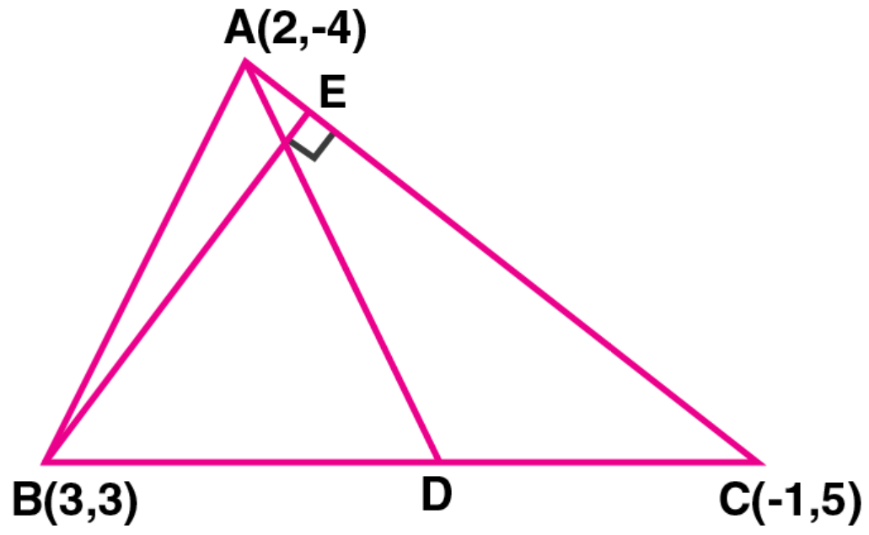Given, A (2, – 4), B (3, 3) and C (– 1, 5) are the vertices of triangle ABC

(i) D is the mid-point of BC

So, the co-ordinates of D will be

((3 – 1)/2, (3 + 5)/2) = (2/2, 8/2) = (1, 4)

Now,

The slope of AC (m1) = (5 + 4)/ (-1 – 2) = 9/-3 = -3

Let the slope of BE be m2

Then, m1 x m2 = -1

-3 x m2 = -1

m2 = 1/3

so, the equation of BE will be

y – 3 = 1/3 (x – 3)

3y – 9 = x – 3

x – 3y + 6 = 0

Thus, the required line equation is x – 3y + 6 = 0.

34. Find the equation of the right bisector of the line segment joining the points (1, 2) and (5, – 6).

Solution:

The slope of the line joining the points (1, 2) and (5, -6) is

m1 = (-6 – 2)/ (5 – 1) = -8/4 = -2

Now, if m2 is the slope of the right bisector of the above line

Then,

m1 x m2 = -1

-2 x m2 = -1

m2 = ½

The mid-point of the line segment joining (1, 2) and (5, -6) will be

((1 + 5)/2, (2 – 6)/2) = (6/2, -4/2) = (3, -2)

So, equation of the line is

y + 2 = ½ (x – 3)

2y + 4 = x – 3

x – 2y – 7 = 0

Thus, the equation of the required right bisector is x – 2y – 7 = 0.

35. Points A and B have coordinates (7, – 3) and (1, 9) respectively. Find

(i) the slope of AB.

(ii) the equation of the perpendicular bisector of the line segment AB.

(iii) the value of ‘p’ if ( – 2, p) lies on it.

Solution:

Given, co-ordinates of points A are (7, -3) and of B are (1, 9)

(i) The slope of AB (m) = (9 + 3)/ (1 – 7) = 12/ (-6) = -2

(ii) Let PQ be the perpendicular bisector of AB intersecting it at M

Now, the co-ordinates of M will be the mid-point of AB

Co-ordinates of M will be

= (7 + 1)/2, (-3 + 9)/2 = 8/2, 6/2

= (4, 3)

The slope of line PQ will be = -1/m = -1/ (-2) = ½

Thus, the equation of PQ is

y – 3 = ½ (x – 4)

2y – 6 = x – 4

x – 2y + 2 = 0

(iii) As point (-2, p) lies on the above line

The point will satisfy the line equation

-2 – 2p + 2 = 0

-2p = 0

p = 0

Thus, the value of p is 0.

36. The points B (1, 3) and D (6, 8) are two opposite vertices of a square ABCD. Find the equation of the diagonal AC.

Solution:

Given, points B (1, 3) and D (6, 8) are two opposite vertices of a square ABCD

Slope of BD is given by

m1 = (8 – 3)/ (6 – 1) = 5/5 = 1

We know that, the diagonal AC is a perpendicular bisector of diagonal BD

So, the slope of AC (m2) will be

m1 x m2 = -1

1 x m2 = -1

m2 = -1

And, the co-ordinates of mid-point of BD and AC will be

((1 + 6)/2 , (3 + 8)/2) = (7/2, 11/2)

So, the equation of AC is

y – 11/2 = -1 (x – 7/2)

2y – 11 = -2x – 7

2x + 2y – 7 – 11 = 0 ⇒ 2x + 2y – 18 = 0

Thus, the equation of diagonal AC is x + y – 9 = 0.

37. ABCD is a rhombus. The co-ordinates of A and C are (3, 6) and ( – 1, 2) respectively. Write down the equation of BD.

Solution:

Given, ABCD is a rhombus and co-ordinates of A are (3, 6) and of C are (-1, 2)

Slope of AC (m1) = (2 – 6)/ (-1 – 3) = -4/-4 = 1

We know that, the diagonals of a rhombus bisect each other at right angles.

So, the diagonal BD is perpendicular to diagonal AC

Let the slope of BD be m2

Then, m1 x m2 = -1

m2 = -1/(m1)

= -1/ (1) = -1

Now, the co-ordinates of the mid-point of AC is given by

((3 – 1)/2, (6 + 2)/2) = (2/2, 8/2) = (1, 4)

So, the equation of BD will be

y – 4 = -1 (x – 1)

y – 4 = -x + 1

x + y = 5

Thus, the equation of BD is x + y = 5.

38. Find the equation of the line passing through the intersection of the lines 4x + 3y = 1 and 5x + 4y = 2 and

(i) parallel to the line x + 2y – 5 = 0

(ii) perpendicular to the x-axis.

Solution:

Given, line equations:

4x + 3y = 1 … (1)

5x + 4y = 2 … (2)

On solving the above equation to find the point of intersection, we have

Multiplying (1) by 4 and (2) by 3

16 x + 12y = 4

15x + 12y = 6

On subtracting, we get

x = -2

Putting the value of x in (1), we have

4(-2) + 3y = 1

-8 + 3y = 1

3y = 1 + 8 = 9

y = 9/3 = 3

Hence, the point of intersection is (-2, 3).

(i) Given line, x + 2y – 5 = 0

2y = -x + 5

y = -(1/2) x + 5/2

Slope (m) = -1/2

A line parallel to this line will have the same slope m = -1/2

So, the equation of line having slope m and passing through (-2, 3) will be

y – 3 = (-1/2) (x + 2)

2y – 6 = -x – 2

x + 2y = 4

(ii) As any line perpendicular to x-axis will be parallel to y-axis.

So, the equation of line will be

x = -2 ⇒ x + 2 = 0

39. (i) Write down the co-ordinates of the point P that divides the line joining A ( – 4, 1) and B (17, 10) in the ratio 1 : 2.

(ii) Calculate the distance OP where 0 is the origin

(iii) In what ratio does the y-axis divide the line AB?

Solution:

(i) Given, co-ordinates of the line joining A ( – 4, 1) and B (17, 10) and point P divides the line segment in the ratio 1 : 2

Let the co- ordinates of P be (x, y)

Then,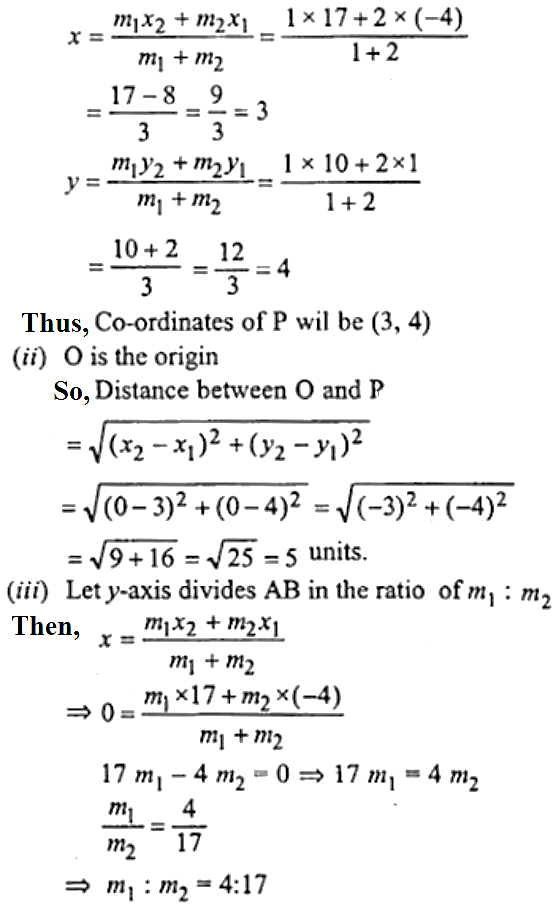40. Find the image of the point (1, 2) in the line x – 2y – 7 = 0.

Solution:

Given line equation: x – 2y – 7 = 0 … (i)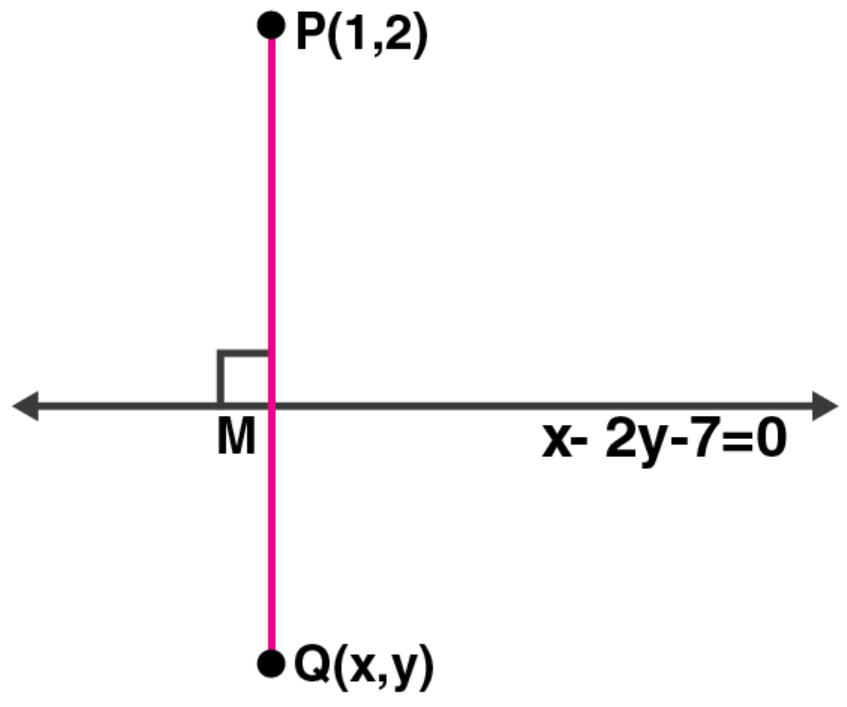Draw a perpendicular from point P (1, 2) on the line

Let P’ be the image of P and let its co-ordinates be (x, y)

The slope of the given line is given as,

2y = x – 7

y = (1/2) x – 7

Slope (m1) = ½

Let the slope of line segment PP’ be m2

As PP’ is perpendicular to the given line, product of slopes: m1 x m2 = -1

So, ½ x m2 = -1

m2 = -2

So, the equation of the line perpendicular to the given line and passing through P (1, 2) is

y – 2 = (-2) (x – 1)

y – 2 = -2x + 2

2x + y – 4 = 0 … (ii)

Let the intersection point of lines (i) and (ii) be taken as M.

Solving both the line equations, we have

Multiplying (ii) by 2 and adding with (i)

x – 2y – 7 = 0

4x + 2y – 8 = 0

———————

5x – 15 = 0

x = 15/5 = 3

Putting value of x in (i), we get

3 – 2y – 7 = 0

2y = -4

y = -4/2 = -2

So, the co-ordinates of M are (3, -2)

Hence, its seen that M should be the mid-point of the line segment PP’

(3, -2) = ((x + 1)/2, (y + 2)/2)

(x + 1)/2 = 3

x + 1 = 6

x = 6 – 1 = 5

And,

(y + 2)/2 = -2

y + 2 = -4

y = -4 – 2 = -6

Therefore, the co-ordinates of P’ are (5, -6).

41. If the line x – 4y – 6 = 0 is the perpendicular bisector of the line segment PQ and the co-ordinates of P are (1, 3), find the co-ordinates of Q.

Solution:

Given, line equation: x – 4y – 6 = 0 … (i)

Co-ordinates of P are (1, 3)

Let the co-ordinates of Q be (x , y)

Now, the slope of the given line is

4y = x – 6

y = (1/4) x – 6/4

slope (m) = ¼

So, the slope of PQ will be (-1/m) [As the product of slopes of perpendicular lines is -1]

Slope of PQ = -1/ (1/4) = -4

Now, the equation of line PQ will be

y – 3 = (-4) (x – 1)

y – 3 = -4x + 4

4x + y = 7 … (ii)

On solving equations (i) and (ii), we get the coordinates of M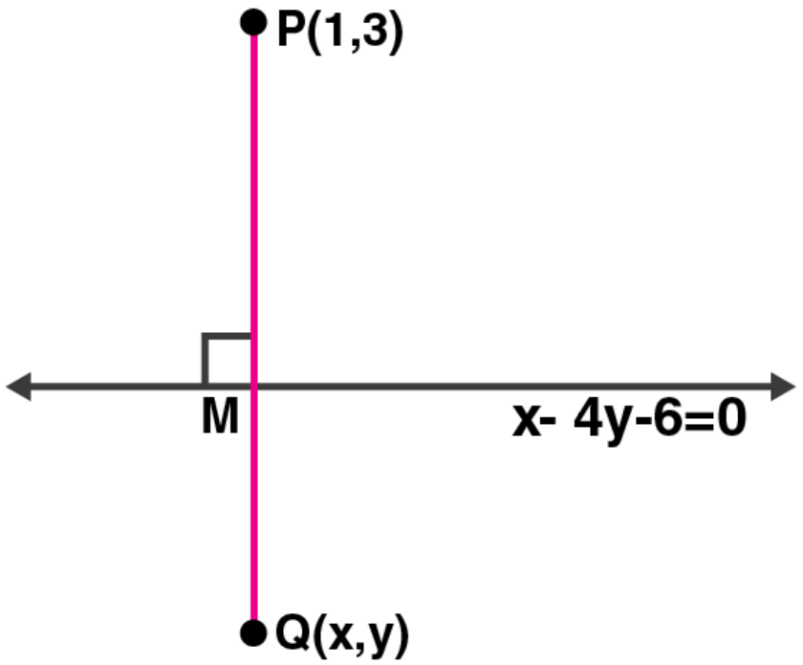Multiplying (ii) by 4 and adding with (i), we get

x – 4y – 6 = 0

16x + 4y = 28

——————

17x = 34

x = 34/17 = 2

Putting the value of x in (i)

2 – 4y – 6 = 0

-4 – 4y = 0

4y = -4

y = -1

So, the co-ordinates of M are (2, -1)

But, M is the mid-point of line segment PQ

(2, -1) = (x + 1)/2 , (y + 3)/2

(x + 1)/2 = 2

x + 1 = 4

x = 3

And,

(y + 3)/2 = -1

y + 3 = -2

y = -5

Thus, the co-ordinates of Q are (3, -5).

42. OABC is a square, O is the origin and the points A and B are (3, 0) and (p, q). If OABC lies in the first quadrant, find the values of p and q. Also write down the equations of AB and BC.

Solution:

Given, OABC is a square

Co-ordinates of A and B are (3, 0) and (p, q) respectively

By distance formula, we have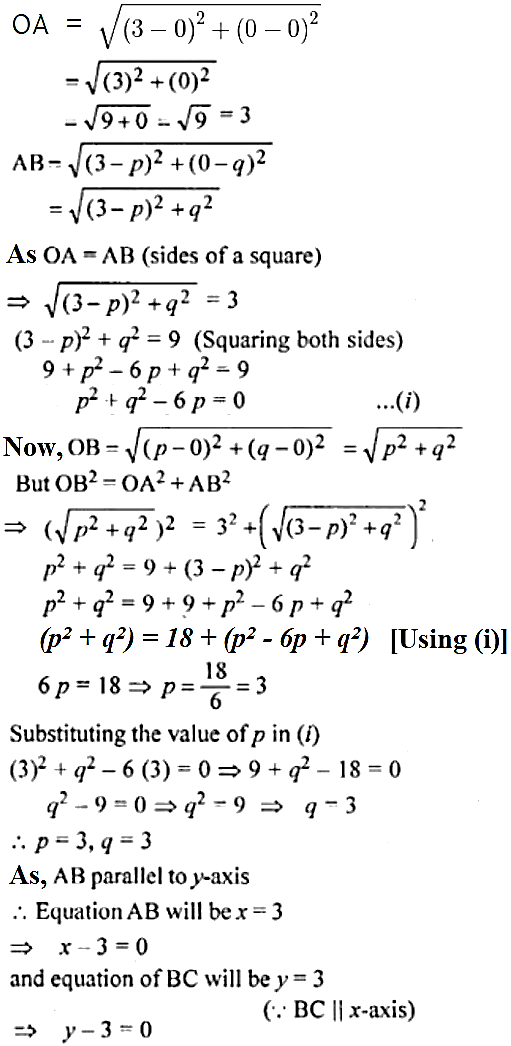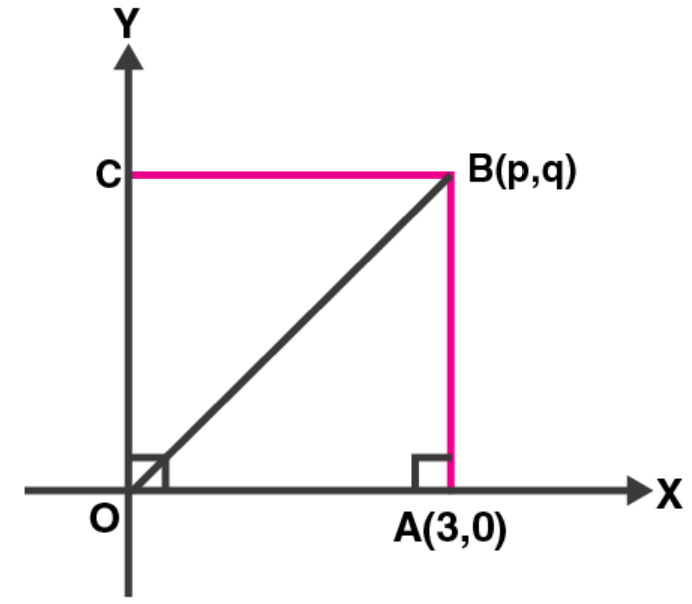Chapter Test

1. Find the equation of a line whose inclination is 60° and y-intercept is – 4.

Solution:

Given, inclination = 60o and y-intercept (c) = -4

So, slope (m) = tan 60o = √3

Hence, the equation of the line is given by

y = mx + c

y = √3x – 4

2. Write down the gradient and the intercept on the y-axis of the line 3y + 2x = 12.

Solution:

Given line equation: 3y + 2x = 12

3y = -2x + 12

y = (-2/3) x + 12/3

y = (-2/3) x + 4

Hence, gradient = -2/3 and the intercept on the y-axis is 4.

3. If the equation of a line is y – √3x + 1, find its inclination.

Solution:

Given line equation: y – √3x + 1

y = √3x – 1

Here, slope = √3

⇒ tan θ = √3

Θ = 60o

Hence, the inclination of the line is 60o.